# JP4875110B2 - Spatial information detection system, detection method thereof, and spatial information detection apparatus - Google Patents

Spatial information detection system, detection method thereof, and spatial information detection apparatus Download PDF

## Info

Publication number
JP4875110B2
JP4875110B2 JP2008557170A JP2008557170A JP4875110B2 JP 4875110 B2 JP4875110 B2 JP 4875110B2 JP 2008557170 A JP2008557170 A JP 2008557170A JP 2008557170 A JP2008557170 A JP 2008557170A JP 4875110 B2 JP4875110 B2 JP 4875110B2
Authority
JP
Japan
Prior art keywords
magnetic field
axis
unit
posture
amplitude
Prior art date
Legal status (The legal status is an assumption and is not a legal conclusion. Google has not performed a legal analysis and makes no representation as to the accuracy of the status listed.)
Active
Application number
JP2008557170A
Other languages
Japanese (ja)
Other versions
JPWO2008096856A1 (en
Inventor

Original Assignee

Priority date (The priority date is an assumption and is not a legal conclusion. Google has not performed a legal analysis and makes no representation as to the accuracy of the date listed.)
Filing date
Publication date
Priority to JP2007030803 priority Critical
Priority to JP2007030803 priority
Priority to JP2007117669 priority
Priority to JP2007117669 priority
Priority to JP2007299759 priority
Priority to JP2007299759 priority
Priority to JP2008557170A priority patent/JP4875110B2/en
Priority to PCT/JP2008/052152 priority patent/WO2008096856A1/en
Application filed by 旭化成エレクトロニクス株式会社 filed Critical 旭化成エレクトロニクス株式会社
Publication of JPWO2008096856A1 publication Critical patent/JPWO2008096856A1/en
Application granted granted Critical
Publication of JP4875110B2 publication Critical patent/JP4875110B2/en
Application status is Active legal-status Critical
Anticipated expiration legal-status Critical

## Images

•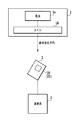•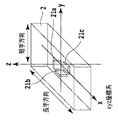•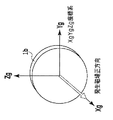•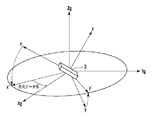•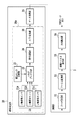•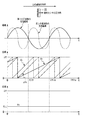•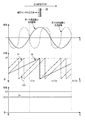•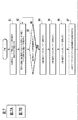•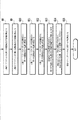•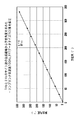•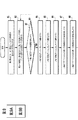•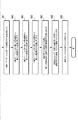•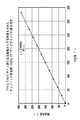•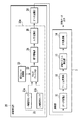•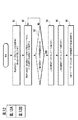•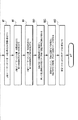•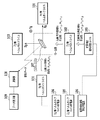•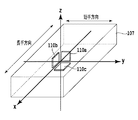•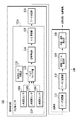•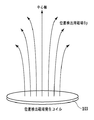•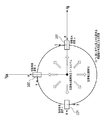•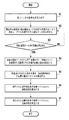•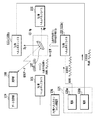•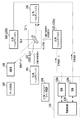•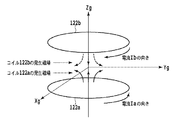•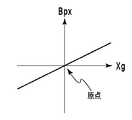•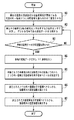•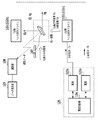••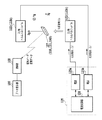•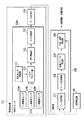•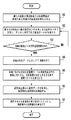•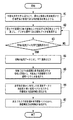•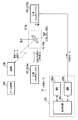•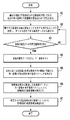•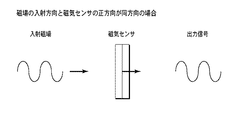•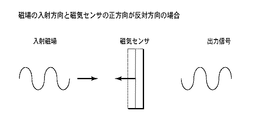## Classifications

• GPHYSICS
• G01MEASURING; TESTING
• G01BMEASURING LENGTH, THICKNESS OR SIMILAR LINEAR DIMENSIONS; MEASURING ANGLES; MEASURING AREAS; MEASURING IRREGULARITIES OF SURFACES OR CONTOURS
• G01B7/00Measuring arrangements characterised by the use of electric or magnetic means
• G01B7/003Measuring arrangements characterised by the use of electric or magnetic means for measuring position, not involving coordinate determination
• GPHYSICS
• G01MEASURING; TESTING
• G01DMEASURING NOT SPECIALLY ADAPTED FOR A SPECIFIC VARIABLE; ARRANGEMENTS FOR MEASURING TWO OR MORE VARIABLES NOT COVERED IN A SINGLE OTHER SUBCLASS; TARIFF METERING APPARATUS; MEASURING OR TESTING NOT OTHERWISE PROVIDED FOR
• G01D5/00Mechanical means for transferring the output of a sensing member; Means for converting the output of a sensing member to another variable where the form or nature of the sensing member does not constrain the means for converting; Transducers not specially adapted for a specific variable
• G01D5/12Mechanical means for transferring the output of a sensing member; Means for converting the output of a sensing member to another variable where the form or nature of the sensing member does not constrain the means for converting; Transducers not specially adapted for a specific variable using electric or magnetic means
• G01D5/14Mechanical means for transferring the output of a sensing member; Means for converting the output of a sensing member to another variable where the form or nature of the sensing member does not constrain the means for converting; Transducers not specially adapted for a specific variable using electric or magnetic means influencing the magnitude of a current or voltage
• G01D5/142Mechanical means for transferring the output of a sensing member; Means for converting the output of a sensing member to another variable where the form or nature of the sensing member does not constrain the means for converting; Transducers not specially adapted for a specific variable using electric or magnetic means influencing the magnitude of a current or voltage using Hall-effect devices
• G01D5/145Mechanical means for transferring the output of a sensing member; Means for converting the output of a sensing member to another variable where the form or nature of the sensing member does not constrain the means for converting; Transducers not specially adapted for a specific variable using electric or magnetic means influencing the magnitude of a current or voltage using Hall-effect devices influenced by the relative movement between the Hall device and magnetic fields

## Description

The present invention relates to a spatial information detection system, a detection method thereof, and a spatial information detection device, and more specifically, can perform continuous measurement using an alternating magnetic field, and has a high degree of freedom in setting a frequency. The present invention relates to a spatial information detection system having a simple configuration, a detection method thereof, and a spatial information detection apparatus.

In recent years, there has been an increasing need for a spatial information detection system that detects the position and orientation of an information terminal. For example, in a space information detection system for detecting the orientation of a movable body such as a head-mounted display in motion capture, or in a medical device field, such as an insertion-type endoscope or a capsule endoscope, where it cannot be seen. A spatial information detection system that measures the direction of an information terminal can be used.

As a spatial information detection method for detecting the position and orientation of these information terminals, there is a method using an alternating magnetic field. At that time, the direction in which the alternating magnetic field is generated and the direction of the magnetic sensor used for measurement become a problem. For example, an AC signal that is measured when the direction in which the output signal increases when a magnetic field enters the magnetic sensor (hereinafter referred to as the positive direction of the magnetic sensor) is the same as the positive direction of the AC magnetic field, and the magnetic sensor The AC signal measured when the positive direction is opposite to the positive direction of the AC magnetic field is apparently indistinguishable.

31A and 31B show the state of the output signal measured when the positive direction of the magnetic sensor is the same direction as the incident direction of the sinusoidal alternating magnetic field (FIG. 31A) and the opposite direction (FIG. 31B). FIG. In both FIG. 31A and FIG. 31B, the output signal is a sine wave and is only in a state shifted by a half wavelength. When the signal is continuously acquired from an appropriate moment, the alternating magnetic field is incident from either direction. I cannot distinguish. Therefore, even if only the signal intensity (amplitude) is detected, the direction of the magnetic sensor cannot be determined, and the amplitude cannot have a positive or negative sign (the sign means that the positive direction of the magnetic sensor is an alternating magnetic field as shown in FIG. 31A). The positive sign (+) is defined as the same as the incident direction, and the negative sign (−) is defined when the positive direction of the magnetic sensor is opposite to the incident direction of the alternating magnetic field as shown in FIG. 31B). That is, the direction of the magnetic sensor cannot be determined based on the sign of the amplitude.

In order to solve such a problem, for example, in Patent Document 1, a magnetic field in which a sine wave A and a sine wave B are superimposed is generated from a generating coil, the magnetic field is measured by a receiving coil, and a sine wave A and a sine wave B Are separated for each frequency band, and the respective signals are synchronously compared to determine the direction in which the generating coil outputs the magnetic field and the direction of the receiving coil.

In the development of a capsule endoscope, for example, as in Non-Patent Document 1, a magnetic field is detected by a receiving coil in synchronization with the generation of an alternating magnetic field in a generating coil, and an FFT (Fast Fourier Transform; Fast Fourier Transform) ) The signal intensity (amplitude) of each frequency is detected by performing an operation, and the phase is calculated from the data from the time of signal detection until the FFT operation is performed. If the same time is used as a reference, the orientation of the magnetic sensor and the magnetic field Since the phase is shifted by π when the direction is the same direction and the opposite direction, the sign of the amplitude of the detected AC magnetic field has been determined.

JP Patent Publication JP Patent Publication JP 2006-214979 A JP 2004-184341 A Japanese Patent Laid-Open No. 11-73600 JP 2003-65791 A JP-A-8-278137 WO2004 / 003476

Biomedical engineering 41-4, 239/249 (2003)

However, the method described in Patent Document 1 described above requires a complicated configuration in which the sine wave B is superimposed intermittently in synchronization with the sine wave A performing positive output. Further, a filter having a predetermined band for separating each frequency is required for each reception coil, and the frequency used for the sine wave A and the sine wave B must be different by 10 times or more. There was a problem that the degree of freedom was low. In addition, the system configuration is such that the generating coil moves, and a power source for generating a magnetic field must be installed in the information terminal, making it difficult to achieve the downsizing and power saving required for recent information terminals. was there.

Further, in the method described in Non-Patent Document 1 described above, in order to detect a phase from the same time, a signal must be measured in synchronization with the generation of an alternating magnetic field, and a trigger (synchronization signal) for that purpose. There is a problem that it is difficult to perform continuous measurement.

In addition, with regard to a spatial information detection device using an AC signal, for example, the width of the vehicle by receiving an AC magnetic field from a direction of a sound wave as in Patent Document 2 or a coil placed at a constant interval as in Patent Document 3 There are also techniques for detecting the position in the direction. In this Patent Document 2, the direction is detected from the phase difference of the waveform received by two elements at different positions, and in Patent Document 3, the position in the width direction is determined from the amplitude of the waveform received by two elements at different positions. However, in both cases, the range of the detection direction is limited, and it is not suitable for detecting the direction of 360 degrees required by recent information terminals.

The present invention has been made in view of such a situation, and the object of the present invention is that continuous measurement can be performed using an alternating magnetic field, and the degree of freedom in setting the frequency is great. Is to provide a simple spatial information detection system, a detection method thereof, and a spatial information detection device.

The present invention has been made to achieve such an object, and the spatial information detection system according to claim 1 of the present invention generates an alternating magnetic field in which the phase relationships of different frequency components are known. A magnetic field generating unit, a magnetic field detecting unit having a multi-axis magnetic sensor for detecting a magnetic field generated from the magnetic field generating unit, and the plurality of axes in each axis based on an output signal of each axis of the magnetic field detecting unit. Based on a Fourier transform unit for calculating the phase and amplitude in the frequency component, and an output signal from the Fourier transform unit, a code is calculated for the amplitude of each axis from the phase relationship of the plurality of frequency components on each axis. And a magnetic field vector calculating unit for calculating a magnetic field vector representing the direction and magnitude of the alternating magnetic field from the sign and the amplitude.

According to a second aspect of the present invention, in the first aspect of the present invention, the posture detection unit that detects the posture of the magnetic field detection unit, an output signal of the posture detection unit, and an output signal of the magnetic field vector calculation unit And a position / posture calculation unit for calculating the posture information and position information of the magnetic field detection unit.

The invention according to claim 3 is the invention according to claim 2, wherein the magnetic field detection unit includes a multi-axis magnetic sensor that detects a DC magnetic field in addition to the AC magnetic field, and the Fourier transform unit. Calculates the amplitude of the DC component in each axis in addition to the phase and amplitude in the plurality of frequency components in each axis, and the magnetic field vector calculation unit adds the magnetic field vector based on the AC magnetic field to the DC A DC magnetic field vector representing the direction and magnitude of the DC magnetic field is calculated from the amplitude of the component, and the position / orientation calculator calculates the attitude of the magnetic field detector from the output signal from the attitude detector and the DC magnetic field vector. Information is calculated, and position information of the magnetic field detection unit is calculated from the posture information and a magnetic field vector based on an alternating magnetic field from the magnetic field generation unit.

The invention according to claim 4 is the invention according to claim 3, wherein the DC magnetic field is geomagnetism.

The invention according to claim 5 is the invention according to any one of claims 1 to 4, wherein the magnetic field generator has a known phase relationship between a plurality of different frequency components, and the direction of the magnetic field or At least one AC inhomogeneous magnetic field having a different magnitude is generated.

The invention according to claim 6 is the invention according to claim 5, characterized in that the non-uniform magnetic field is an alternating gradient magnetic field in which the phase relationship of a plurality of different frequency components is known.

According to a seventh aspect of the present invention, in the first aspect of the invention, the magnetic field generator includes an alternating current uniform magnetic field in which the phase relationship between different frequency components is known, and a plurality of different frequency components. An alternating inhomogeneous magnetic field having a known phase relationship and a different magnetic field direction or magnitude depending on the position is generated, and the magnetic field detecting unit detects the uniform magnetic field and the inhomogeneous magnetic field, and the magnetic field vector The calculation unit calculates a sign for the amplitude of each axis with respect to the uniform magnetic field and the non-uniform magnetic field from the phase relationship of the plurality of frequency components of each axis based on the output signal from the Fourier transform unit. Calculating a uniform magnetic field vector and a non-uniform magnetic field vector representing the directions and magnitudes of the uniform magnetic field and the non-uniform magnetic field from the amplitude and the sign of each axis, and the magnetic field vector calculating unit The attitude information of the magnetic field detection unit is calculated from the output uniform magnetic field vector, and the position information of the magnetic field detection unit is calculated from the attitude information and the nonuniform magnetic field vector output from the magnetic field vector calculation unit. It has a position / attitude calculation unit.

The invention according to claim 8 is the invention according to claim 7, further comprising a posture detection unit that detects a posture of the magnetic field detection unit, wherein the position / posture calculation unit outputs from the posture detection unit. And attitude information of the magnetic field detection unit from the uniform magnetic field vector output from the magnetic field vector calculation unit, and the posture information and the inhomogeneous magnetic field vector output from the magnetic field vector calculation unit The position information of the magnetic field detector is calculated.

The invention according to claim 9 is the invention according to claim 7, wherein the magnetic field detector includes a multi-axis magnetic sensor that detects a DC magnetic field in addition to the uniform magnetic field and the non-uniform magnetic field. The Fourier transform unit calculates the amplitude of the DC component in each axis in addition to the phase and amplitude in the plurality of frequency components in each axis, and the magnetic field vector calculation unit calculates the uniform magnetic field vector and the non-uniformity. In addition to the uniform magnetic field vector, a DC magnetic field vector representing the direction and magnitude of the DC magnetic field is calculated from the amplitude of the DC component, and the position / attitude calculation unit outputs the uniform magnetic field vector output from the magnetic field vector calculation unit. And attitude information of the magnetic field detector from the DC magnetic field vector, and the magnetic field from the attitude information and the inhomogeneous magnetic field vector output from the magnetic field vector calculator. And calculates the position information of the detection unit.

The invention according to claim 10 is the invention according to claim 9, wherein the DC magnetic field is geomagnetism.

The invention according to claim 11 is the invention according to any one of claims 7 to 10, wherein the inhomogeneous magnetic field is an alternating gradient magnetic field in which the phase relationship of different frequency components is known. It is characterized by.

According to a twelfth aspect of the present invention, in the invention according to any one of the seventh to eleventh aspects, the magnetic field generator includes a coil that generates the superposed uniform magnetic field and the non-uniform magnetic field. It is characterized by that.

The invention according to claim 13 is the invention according to any one of claims 1 to 12, wherein an integer ratio of the plurality of frequency components is an even number to an odd number.

The invention according to claim 14 is the invention according to claim 13, wherein the integer ratio is 2: 1.

In addition, the spatial information detection method according to claim 15 of the present invention uses a magnetic field detection unit having a multi-axis magnetic sensor to detect an alternating magnetic field in which the phase relationship of a plurality of different frequency components is known. Step, a Fourier transform step for calculating phases and amplitudes in the plurality of frequency components in each axis based on the output signal of each axis from the magnetic field detection step, and an output signal from the Fourier transform step Calculating a sign for the amplitude of each axis from the phase relationship of the plurality of frequency components of each axis, and calculating a magnetic field vector representing the direction and magnitude of the AC magnetic field from the sign and the amplitude And a step.

According to a sixteenth aspect of the present invention, in the fifteenth aspect of the present invention, an attitude detection step for detecting an attitude of the magnetic field detection unit, an output signal of the attitude detection step, and an output signal of the magnetic field vector calculation step And a position / posture calculation step for calculating the posture information and the position information of the magnetic field detection unit.

The invention according to claim 17 is the invention according to claim 16, wherein the magnetic field detection step detects a direct-current magnetic field in addition to the alternating-current magnetic field, and the Fourier transform step includes the plurality of the plurality of axes on each axis. In addition to the phase and amplitude in the frequency component, the amplitude of the DC component in each axis is calculated, and the magnetic field vector calculating step adds the magnetic field vector based on the AC magnetic field and the amplitude of the DC magnetic field from the amplitude of the DC component. A DC magnetic field vector representing a direction and a magnitude is calculated, and the position / posture calculation step calculates posture information of the magnetic field detection unit from the output signal from the posture detection step and the DC magnetic field vector, and the posture information And position information of the magnetic field detector is calculated from the magnetic field vector based on the alternating magnetic field.

According to an eighteenth aspect of the present invention, in the invention of the fifteenth aspect, the magnetic field detecting step includes an AC uniform magnetic field in which a phase relationship between a plurality of different frequency components is known, and a plurality of different frequency components. An AC inhomogeneous magnetic field having a known phase relationship and a magnetic field direction or magnitude depending on a position is detected, and the magnetic field vector calculation step is performed based on an output signal from the Fourier transform step. From the phase relationship of the plurality of frequency components, in addition to the uniform magnetic field, a sign is calculated for the amplitude of each axis with respect to the non-uniform magnetic field, and the directions of the uniform magnetic field and the non-uniform magnetic field are calculated from the amplitude and sign of each axis. And calculating a uniform magnetic field vector and a non-uniform magnetic field vector representing the magnitude, and calculating the magnetic field from the uniform magnetic field vector output from the magnetic field vector calculating step. A position / posture calculation step for calculating posture information of the detection unit, and calculating position information of the magnetic field detection unit from the posture information and the non-uniform magnetic field vector output from the magnetic field vector calculation step. And

The invention described in claim 19 is the invention described in claim 18, further comprising an attitude detection step for detecting an attitude of the magnetic field detector, wherein the position / orientation calculation step is different from the attitude detection step. The attitude information of the magnetic field detection unit is calculated from the output and the uniform magnetic field vector output from the magnetic field vector calculation unit, and from the attitude information and the inhomogeneous magnetic field vector output from the magnetic field vector calculation step The position information of the magnetic field detector is calculated.

The invention according to claim 20 is the invention according to claim 18, wherein the magnetic field detection step detects a DC magnetic field in addition to the uniform magnetic field and the non-uniform magnetic field, and the Fourier transform step comprises In addition to the phase and amplitude in the plurality of frequency components in each axis, the amplitude of the DC component in each axis is calculated, and the magnetic field vector calculation step adds the uniform magnetic field vector and the inhomogeneous magnetic field vector to the direct current A DC magnetic field vector representing the direction and magnitude of the DC magnetic field is calculated from the amplitude of the component, and the position / orientation calculating step is based on the uniform magnetic field vector output from the magnetic field vector calculating step and the DC magnetic field vector. Calculating the attitude information of the magnetic field detection unit, and the inhomogeneous magnetic field vector output from the attitude information and the magnetic field vector calculation unit And calculates the position information of the magnetic field detecting unit from.

A spatial information detection device according to claim 21 of the present invention is a multi-axis magnetic sensor that detects a magnetic field generated from a magnetic field generation unit that generates an alternating magnetic field in which the phase relationships of different frequency components are known. A magnetic field detection unit having a plurality of frequency components in each axis based on an output signal of each axis of the magnetic field detection unit, and an output from the Fourier transform unit Based on the signal, a sign is calculated for the amplitude of each axis from the phase relationship of the plurality of frequency components of each axis, and a magnetic field vector representing the direction and magnitude of the AC magnetic field is calculated from the sign and the amplitude. And a magnetic field vector calculation unit.

According to a twenty-second aspect of the present invention, in the twenty-first aspect of the present invention, an attitude detection unit that detects an attitude of the magnetic field detection unit, an output signal of the attitude detection unit, and an output signal of the magnetic field vector calculation unit And a position / posture calculation unit for calculating the posture information and position information of the magnetic field detection unit.

The invention according to claim 23 is the invention according to claim 22, wherein the magnetic field detector includes a multi-axis magnetic sensor that detects a DC magnetic field in addition to the AC magnetic field, and the Fourier transform unit. Calculates the amplitude of the DC component in each axis in addition to the phase and amplitude in the plurality of frequency components in each axis, and the magnetic field vector calculation unit adds the magnetic field vector based on the AC magnetic field to the DC A DC magnetic field vector representing the direction and magnitude of the DC magnetic field is calculated from the amplitude of the component, and the position / orientation calculator calculates the output of the magnetic field detector from the output signal from the attitude detector and the DC magnetic field vector. Attitude information is calculated, and position information of the magnetic field detector is calculated from the attitude information and a magnetic field vector based on the alternating magnetic field.

According to a twenty-fourth aspect of the present invention, in the invention of the twenty-first aspect, the magnetic field detection unit includes an AC uniform magnetic field in which the phase relationship between different frequency components is known and a plurality of different frequency components. A magnetic field generated from a magnetic field generation unit that generates an alternating inhomogeneous magnetic field having a known phase relationship and a magnetic field direction or magnitude depending on a position is detected, and the magnetic field vector calculation unit is Based on the output signal, a sign is calculated for the amplitude of each axis relative to the non-uniform magnetic field in addition to the uniform magnetic field from the phase relationship of the plurality of frequency components of each axis, and the amplitude of each axis and A uniform magnetic field vector and a non-uniform magnetic field vector representing the direction and magnitude of the uniform magnetic field and the non-uniform magnetic field are calculated from the code, and output from the magnetic field vector calculation unit Position / orientation calculation for calculating attitude information of the magnetic field detection unit from one magnetic field vector, and calculating position information of the magnetic field detection unit from the attitude information and the non-uniform magnetic field vector output from the magnetic field vector calculation unit It comprises a part.

An invention according to claim 25 is the invention according to claim 24, further comprising an attitude detection unit that detects an attitude of the magnetic field detection unit, wherein the position / orientation calculation unit outputs from the attitude detection unit. And attitude information of the magnetic field detection unit from the uniform magnetic field vector output from the magnetic field vector calculation unit, and the posture information and the inhomogeneous magnetic field vector output from the magnetic field vector calculation unit The position information of the magnetic field detector is calculated.

According to a twenty-sixth aspect of the invention, in the invention of the twenty-fourth aspect, the magnetic field detector includes a multi-axis magnetic sensor that detects a DC magnetic field in addition to the uniform magnetic field and the non-uniform magnetic field. The Fourier transform unit calculates the amplitude of the DC component in each axis in addition to the phase and amplitude in the plurality of frequency components in each axis, and the magnetic field vector calculation unit calculates the uniform magnetic field vector and the non-uniformity. In addition to the uniform magnetic field vector, a DC magnetic field vector representing the direction and magnitude of the DC magnetic field is calculated from the amplitude of the DC component, and the position / attitude calculation unit outputs the uniform magnetic field vector output from the magnetic field vector calculation unit. And attitude information of the magnetic field detector from the DC magnetic field vector, and from the attitude information and the inhomogeneous magnetic field vector output from the magnetic field vector calculator. And calculates the position information of the magnetic field detecting portion.

According to the present invention, a magnetic field generation unit that generates a magnetic field in which the phase relationship of a plurality of different frequency components is known, and a magnetic field detection unit that includes a multi-axis magnetic sensor that detects the magnetic field generated from the magnetic field generation unit, Based on the output signal of each axis of the magnetic field detection unit, a Fourier transform unit that calculates the phase and amplitude in a plurality of frequency components in each axis, and on the basis of the output signal from the Fourier transform unit, Since a sign is calculated for the amplitude of each axis from the phase relationship of a plurality of frequency components, and a magnetic field vector calculation unit for calculating a magnetic field vector representing the direction and magnitude of the magnetic field from the sign and the amplitude, an AC magnetic field is provided. It is possible to provide a spatial information detection system that can perform continuous measurement using, has a high degree of freedom in frequency setting, and has a simple configuration. Similarly, the spatial information detection method and the spatial information detection device can be provided.

It is a whole block diagram which shows Embodiment 1 of the spatial information detection system of this invention. It is a figure which shows the coil coordinate system of the information terminal and magnetic field generation | occurrence | production part in the spatial information detection system of this invention, and is a figure which shows the coordinate system of an information terminal. It is a figure which shows the coil coordinate system of the information terminal and magnetic field generation | occurrence | production part in the spatial information detection system of this invention, and is a figure which shows the coordinate system of the coil of a magnetic field generation | occurrence | production part. It is a figure which shows the relationship between the direction of the information terminal in a spatial information detection system of this invention, and a coordinate system. It is a concrete block diagram of the configuration of the spatial information detection device of the first embodiment in the spatial information detection system of the present invention. It is a figure which shows the relationship between the direction of a magnetic sensor, and the direction of the alternating magnetic field which is generate | occur | producing, and the phase relationship of a 1st frequency component and a 2nd frequency component. It is another figure which shows the relationship between the direction of a magnetic sensor, and the direction of the alternating magnetic field which has generate | occur | produced, and the phase relationship of a 1st frequency component and a 2nd frequency component. It is a figure which shows the flowchart for demonstrating operation | movement of Embodiment 1 of the spatial information detection system of this invention. It is a figure which shows the flowchart for demonstrating operation | movement of Embodiment 1 of the spatial information detection system of this invention. It is a figure which shows the result of the simulation assumed that the magnetic field which superimposed the sine wave magnetic field of 1 Hz and 2 Hz was measured by rotating an azimuth angle sensor 30 degree | times horizontally with a magnetic field. It is a figure which shows the flowchart for demonstrating operation | movement of Embodiment 2 of the spatial information detection system of this invention. It is a figure which shows the flowchart for demonstrating operation | movement of Embodiment 2 of the spatial information detection system of this invention. It is a figure which shows the result of the simulation assumed that the magnetic field which overlap | superposed the sinusoidal magnetic field of 3 Hz and 8 Hz was measured by rotating an azimuth angle sensor 30 degree | times horizontally with a magnetic field. It is a concrete block diagram of the spatial information detection apparatus of Embodiment 3 in the spatial information detection system of this invention. It is a figure which shows the flowchart for demonstrating operation | movement of Embodiment 3 of the spatial information detection system of this invention. It is a figure which shows the flowchart for demonstrating operation | movement of Embodiment 3 of the spatial information detection system of this invention. It is a whole block diagram which shows Embodiment 4 of the spatial information detection system of this invention. It is explanatory drawing about the coordinate system of an information terminal. It is a concrete block diagram of the spatial information detection apparatus of Embodiment 4 in the spatial information detection system of this invention. It is a figure which shows typically regarding the direction of the magnetic field which the magnetic field Bp for position detection makes. It is a figure which shows a mode that the magnetic field Bp for position detection from a position detection magnetic field generation coil was seen from Zg-axis upper direction. It is a figure which shows the flowchart for demonstrating operation | movement of Embodiment 4 of the spatial information detection system of this invention. It is a whole block diagram which shows Embodiment 5 of the spatial information detection system of this invention. It is a whole block diagram which shows Embodiment 6 of the spatial information detection system of this invention. It is explanatory drawing about a gradient magnetic field generation | occurrence | production mechanism. It is explanatory drawing about a gradient magnetic field generation | occurrence | production mechanism. It is a figure which shows the flowchart for demonstrating operation | movement of Embodiment 6 of the spatial information detection system of this invention. It is a whole block diagram which shows Embodiment 7 of the spatial information detection system of this invention. It is a figure which shows the flowchart for demonstrating operation | movement of Embodiment 7 of the spatial information detection system of this invention. It is a whole block diagram which shows Embodiment 8 of the spatial information detection system of this invention. It is a concrete block diagram of the spatial information detection apparatus of Embodiment 8 in the spatial information detection system of this invention. It is a figure which shows the flowchart for demonstrating operation | movement of Embodiment 8 of the spatial information detection system of this invention. It is a figure which shows the flowchart for demonstrating operation | movement of Embodiment 9 of the spatial information detection system of this invention. It is a whole block diagram which shows Embodiment 10 of the spatial information detection system of this invention. It is a figure which shows the flowchart for demonstrating operation | movement of Embodiment 10 of the spatial information detection system of this invention. It is a figure which shows the mode of the output signal measured when the positive direction of a magnetic sensor is the same direction with respect to the incident direction of a sinusoidal AC magnetic field. It is a figure which shows the mode of the output signal measured when the positive direction of a magnetic sensor is the opposite direction with respect to the incident direction of a sinusoidal AC magnetic field.

Hereinafter, embodiments of the present invention will be described with reference to the drawings.
In the present invention, spatial information means posture or position information. The posture may be a part of the posture with a degree of freedom remaining, or may be an arbitrary posture with no degree of freedom left.

First, detection of a direction that is a partial posture will be described below.
For detection of this direction, when a triaxial magnetic sensor is used at a generated magnetic field frequency ratio 1: 2 (embodiment 1), or when a triaxial magnetic sensor is used at a generated magnetic field frequency ratio M: N (embodiment 1). 2) There are cases where a biaxial magnetic sensor is used at a frequency ratio M: N of the generated magnetic field (Embodiment 3). Each of these embodiments will be described below. In the first to third embodiments, the generated magnetic field is described as a magnetic field generated from one coil. The magnetic field generated from one coil spreads symmetrically about the central axis of the coil. In the first to third embodiments, a method for detecting the direction of an information terminal within a range that can be regarded as a magnetic field generated in one direction is described. To do. That is, in the embodiment, the information terminal is in a region that can be treated as a uniform magnetic field in which the direction and magnitude of the magnetic field can be regarded as constant, or on the central axis of the coil or in a fixed position.

[Embodiment 1]
<When using a 3-axis magnetic sensor with a generated magnetic field frequency ratio of 1: 2>
FIG. 1 is an overall configuration diagram showing Embodiment 1 in the spatial information detection system of the present invention, in which reference numeral 1 is a magnetic field generator, 1a is a power source, 1b is a coil, 2 is an information terminal, 20 is a magnetic field detector, Reference numeral 21 denotes a magnetic sensor, and 3 denotes a calculation unit. The first embodiment includes one magnetic field generation unit with a frequency ratio of 1: 2, and the magnetic field detection unit 20 includes a triaxial magnetic sensor 21.

The spatial information detection system of the present invention includes a magnetic field generation unit 1, a magnetic field detection unit 20, a Fourier transform unit (reference numeral 32 in FIG. 4 to be described later), a magnetic field vector calculation section (reference numeral 33 in FIG. 4 to be described later), It is comprised from the calculating part 3 which has a direction calculation part (code | symbol 34 of FIG. 4 mentioned later). The magnetic field detection unit 20 is attached to the information terminal 2 whose direction is to be detected.

The information terminal 2 means a part or part from which a user can obtain some information, such as a mobile phone, a PDA (Personal Digital Assistant), a capsule endoscope, an endoscope, a game machine. It means various things. The calculation unit 3 is, for example, a mobile phone, a PDA, a game machine, a capsule endoscope, a measuring instrument for an endoscope, or a CPU (Central Processing Unit) in a PC (Central Processing Unit). A processing device), a DSP (Digital Signal Processor), a microcomputer, and the like are configured using a storage device such as a memory and a hard disk, a communication function with the outside, and the like.

The magnetic field generator 1 that constitutes the spatial information detection system of the present invention generates an alternating magnetic field in which the phase relationships of different frequency components are known. As shown in FIG. 1, the power source 1a includes a coil 1b, and the power source 1a can generate two alternating currents (or voltages) having different frequencies in a superimposed manner. The angular frequencies are ω and 2ω, respectively (the frequency ratio is 1: 2 as the smallest integer ratio). A magnetic field can be generated by applying a generated current from the power source 1a to the coil 1b. The phase of the generated AC magnetic field is assumed to be −π / 2 at time t = 0 for both the first frequency component and the second frequency component. That is, if the phase Θ of the magnetic field of the first frequency component is Φ and the phase of the magnetic field of the second frequency component is Φ,
Θ = ωt−π / 2 (1)
Φ = 2ωt−π / 2 (2)
It can be expressed as

The specific configuration of the magnetic field detection unit 20 is shown in FIG. 4, but a three-axis magnetic sensor 21 that detects the magnetic field generated from the magnetic field generation unit 1 (X-axis magnetic sensors 21a, Y in FIG. 4). Includes an azimuth angle sensor 20a equipped with an axial magnetic sensor 21b and a Z-axis magnetic sensor 21c). The azimuth angle sensor 20a is equipped with three-axis magnetic sensors 21a, 21b, 21c facing mutually orthogonal planes. ing. This magnetic sensor is, for example, a semiconductor type magnetic sensor such as a Hall element, MR element, GMR element, or MI element.

Although the specific configuration of the calculation unit 3 is shown in FIG. 4, the calculation unit 3 includes a Fourier transform unit 32, a magnetic field vector calculation unit 33, and a direction calculation unit 34. It may or may not be installed. In addition, although the signal line is drawn between the information terminal 2 and the calculating part 3 in FIG. 1, it may be wireless.

As will be described later with reference to FIG. 4, the Fourier transform unit 32 calculates the phase and amplitude in a plurality of frequency components on each axis based on the output signal of each axis of the magnetic field detection unit 20. As will be described later with reference to FIG. 4, the magnetic field vector calculation unit 33 calculates a code for the amplitude of each axis from the phase relationship of the plurality of frequency components of each axis based on the output signal from the Fourier transform unit 32. A magnetic field vector representing the direction and magnitude of the magnetic field is calculated from the sign of each axis and the amplitude of at least one frequency component of a plurality of frequency components. The direction calculation unit 34 calculates the direction in which the information terminal 2 is facing based on the output from the magnetic field vector calculation unit 33.

2A and 2B are diagrams showing the coordinate system of the information terminal and the magnetic field generator coil in the spatial information detection system of the present invention. FIG. 2A shows the coordinate system of the information terminal, and FIG. 2B shows the coil of the magnetic field generator. It is a figure which shows each coordinate system.

As shown in FIG. 2A, the longitudinal direction of the information terminal 2 is the x axis, the short side direction is the y axis, and the z axis perpendicular to the x axis and the y axis is a right-handed coordinate system xyz coordinate system (terminal coordinate system). Define In addition, it is assumed that the directions of the three-axis magnetic sensors 21a, 21b, and 21c that the azimuth angle sensor 20a of the information terminal 2 has are coincident with the directions of the axes that constitute the xyz coordinate system. That is, there are x-axis, y-axis, and z-axis magnetic sensors, and the increasing direction (positive direction) of each output is the positive direction of each axis of the xyz coordinate system.

Further, as shown in FIG. 2B, the coil 1b is installed vertically on the horizontal plane, and the Xg axis coincides with the direction of the positive magnetic field generated from the coil 1b, and vertically upwards with respect to the horizontal plane (ground plane). The right-handed coordinate system XgYgZg coordinate system (absolute coordinate system) is composed of the Zg axis that faces and the Yg axis that forms a plane parallel to the horizontal plane as the Xg axis.

FIG. 3 is a diagram showing the relationship between the direction of the information terminal and the coordinate system in the spatial information detection system of the present invention. In FIG. 3, the xyz coordinate system and the XgYgZg coordinate system of the information terminal 2 facing in a certain direction do not match except for the origin. The direction data Ψ, which is the spatial information of the information terminal 2 detected by the spatial information detection system of the first embodiment, is obtained by converting the x-axis and y-axis of the information terminal 2 into the XgYg plane, and the vectors when the coordinate data are converted to the x′-axis and y-axis, respectively. When expressed as the “axis”, it is represented by an angle between the x ′ axis and the Xg axis, which is the generation direction of the positive magnetic field. (First, rotate around the x axis, move the y axis to the y ′ axis, then rotate around the y ′ axis, and move the x axis to the x ′ axis.) The direction data as shown in FIG. Ψ is also called an azimuth angle. The direction data ψ detected by the spatial information detection system according to the first embodiment of the present invention is not limited to the azimuth angle depending on the application, and may be a magnetic field vector representing the direction of the magnetic field detected by the information terminal 2.

FIG. 4 is a block diagram showing a specific configuration of the spatial information detection apparatus according to the first embodiment in the spatial information detection system of the present invention. This spatial information detection apparatus includes a magnetic field detection unit 20 and a calculation unit 3, and the calculation unit 3 includes a data reception unit 31, a Fourier transform unit 32, a magnetic field vector calculation unit 33, and a direction calculation unit 34. . The magnetic field detection unit 20 includes an azimuth angle sensor 20 a and a data transmission unit 26.

The azimuth angle sensor 20a is configured by selecting a three-axis magnetic sensor 21 including an x-axis magnetic sensor 21a, a y-axis magnetic sensor 21b, and a z-axis magnetic sensor 21c, and selecting the three-axis magnetic sensor 21. A multiplexer unit 22 for obtaining an output signal from the magnetic sensor; a magnetic sensor driving unit 23 for driving the magnetic sensor 21 via the multiplexer unit 22; a signal amplifying unit 24 for amplifying an output signal from the multiplexer unit 22; The A / D converter 25 is configured to A / D convert the amplified signal from the signal amplifier 24. The data transmission unit 26 transmits the signal converted by the A / D conversion unit 25 to the calculation unit 3.

With such a configuration, the magnetic sensor driving unit 23 drives the magnetic sensor 21 via the multiplexer unit 22. The multiplexer unit 22 selects the magnetic sensor of the axis to be measured. The signal of the magnetic sensor on the selected axis is amplified to an appropriate magnitude by the signal amplifier 24 and converted from an analog signal to a digital signal by the A / D converter 25. This conversion into a digital signal is performed on the signal from the magnetic sensor of each axis. The digitized signal is transmitted from the data transmission unit 26 to the calculation unit 3 as magnetic data.

As described above, the calculation unit 3 includes the data reception unit 31 that receives magnetic data from the data transmission unit 26 of the magnetic field detection unit 20, the Fourier transform unit 32, the magnetic field vector calculation unit 33, and the direction calculation unit 34. It is composed of

The Fourier transform unit 32 calculates the phase and amplitude in a plurality of frequency components on each axis from the output signal from the data receiving unit 31. In addition, the magnetic field vector calculation unit 33 calculates a code for the amplitude of each axis from the phase relationship of a plurality of frequency components of each axis from the output signal from the Fourier transform unit 32, and calculates the direction and magnitude of the magnetic field from the code and the amplitude. Calculate the magnetic field vector to represent. Further, the direction calculation unit 34 calculates the direction of the information terminal 2 from the output signal from the magnetic field vector calculation unit 33.

With such a configuration, the data receiving unit 31 receives the magnetic data transmitted from the data transmitting unit 26 of the magnetic field detecting unit 20 and sends it to the Fourier transform unit 32. The Fourier transform unit 32 acquires a desired amount of magnetic data from the triaxial magnetic sensor 21, and then performs an FFT operation (fast Fourier transform) on the magnetic data. The data amount acquired as the desired amount is, for example, a data amount for an integer period of the waveform of the superimposed alternating magnetic field. For example, when the magnetic field data is obtained by superimposing the alternating magnetic fields of 1 Hz and 2 Hz and acquiring the magnetic data by sampling at 128 Hz by the azimuth sensor 20a, the data amount in which the period of 1 Hz and 2 Hz is an integer multiple is 128. Is the amount of data to be acquired. Execution of the FFT operation is performed separately on the x-axis desired amount of magnetic data, the y-axis desired amount of magnetic data, and the z-axis desired amount of magnetic data. A method may be used in which a complex FFT operation is performed on a certain amount of magnetic data to obtain FFT data, and an FFT operation is separately performed on the remaining one-axis desired amount of magnetic data. Then, the amplitude of each axis of the first frequency component and the phase of the first and second frequency components are calculated from the FFT data of each axis calculated by performing the FFT operation, and sent to the magnetic field vector calculation unit 33. .

Here, from the FFT data of each axis, the amplitude calculated by the Fourier transform unit 32 is A x , A y , A z on the x axis, y axis, and z axis, respectively, and the phase of the calculated first frequency component Are represented as θ x , θ y , θ z , and the respective axis components of the phase of the second frequency component are represented as φ x , φ y , φ z (0 ≦ θ x , θ y , θ z , φ x , φ y , φ z <2π).

These calculated phases are sent to the magnetic field vector calculation unit 33, and the amplitude code obtained from the following phase relationship indicates which direction the positive direction of the magnetic sensor of the measured axis is directed to the positive direction of the magnetic field. The sign of the amplitude is determined using the determination value. The x-axis component will be described below.

The amplitude code determination value η x is changed to η x = φ x −2 × θ x (Expression 3)
It is defined as However, 0 ≦ η x <2π.

When the positive direction of the magnetic sensor 21 and the positive direction of the generated magnetic field coincide with each other, if the phase of the calculated first frequency component and second frequency component is θ x + and φ x + ,
θ x + = ωt−π / 2-2πp x + (Expression 4)
φ x + = 2ωt−π / 2-2πq x + (Formula 5)
(However, p x + and q x + are integers for satisfying 0 ≦ θ x + and φ x + <2π). And the amplitude code determination value η x + at that time is from (Equation 3),
η x + = π / 2 + 2π (2p x + −q x + ) −2πν x + (Expression 6)
And is a constant value (where ν x + is an integer for making 0 ≦ η x + <2π). On the other hand, when the positive direction of the magnetic sensor 21 and the positive direction of the generated magnetic field are opposite, if the phases of the calculated first frequency component and second frequency component are θ x and φ x ,
θ x = ωt−π / 2 + π−2πp x (Expression 7)
φ x = 2ωt−π / 2 + π−2πq x (Expression 8)
(The phase is shifted by π. Also, p x and q x are integers for setting 0 ≦ θ x and φ x <2π). In this case, the amplitude code determination value η x is ( from equation 3) η x - = 3π / 2 + 2π (2p x - -q x -) -2πν x - ··· ( equation 9)
(Where ν x is an integer for setting 0 ≦ η x <2π), which is also a constant value.

Therefore, the amplitude sign decision value eta x calculated from (Equation 3) is eta x (Formula 6) of eta x + and (9) - as, from the different values of the generated magnetic field direction and the magnetic sensor 21 It can be determined whether the orientation relationship is the same direction or the opposite direction. It is possible to determine the sign of the amplitude.

For example, the sign of the amplitude of the x-axis Sign (A x ) (where Sign (k) represents the sign of k and becomes −1 or +1) can be expressed by using η x in (Equation 3) to sign (A x ) = Sign (Sin (η x )) (Equation 10)
Can be expressed as

5 and 6 are diagrams showing the relationship between the direction of the magnetic sensor and the direction of the generated alternating magnetic field, and the phase relationship between the first frequency component and the second frequency component. The relationship between the direction of the positive magnetic field in the uppermost stage and the positive direction of the magnetic sensor 21 is measured by the magnetic sensor 21 in the second stage. The fluctuation of the alternating magnetic field is measured from t = 0 to t = 3π / ω for each frequency component. In the figure, the phase θ x of the first frequency component, the phase φ x of the second frequency component of the second stage AC magnetic field in the third stage, and the time variation of 2θ x are represented by a solid line, a broken line, and a one-dot chain line, respectively. = 0 to t = 3π / ω, and the time course of η x (= φ x −2θ x ) is shown at the bottom stage from t = 0 to t = 3π / ω.

FIG. 5 shows the case where the positive direction of the magnetic field and the positive direction of the magnetic sensor are the same direction, and FIG. 6 shows the case of the opposite direction. In FIG. 5, η x is constant at π / 2 (corresponding to η x + in (Expression 6)), and in FIG. 6 η x is constant at 3π / 2 (corresponding to η x in (Expression 9)). Become. In the figure, the magnetosensitive surface (surface for detecting the magnetic field) of the magnetic sensor 21 is shown as being perpendicular to the magnetic field. However, when the magnetic sensor 21 is not perpendicular, the amplitude of the magnetic field to be measured is reduced, and the magnetosensitive surface becomes the magnetic field. Η x + and η x in (Expression 6) and (Expression 9) do not change until they are parallel and face in the opposite direction. Therefore, it is possible to determine whether the output of the magnetic sensor 21 is positive or negative. As seen above, η x + and η x in (Equation 6) and (Equation 9) take time-independent values, and the phase calculation from data synchronized with the magnetic field is used to determine the sign of the amplitude. Therefore, the measurement timing can be freely selected, and continuous measurement is possible. And there exists an advantage that it is not necessary to utilize the structure which superimposes a signal intermittently like patent document 1 mentioned above.

Similarly to the x-axis, the signs of the y-axis and z-axis amplitudes are obtained from the y-axis and z-axis amplitude sign determination values η y and η z , and the next signed amplitude is calculated. If the signed amplitudes of the x-axis, y-axis, and z-axis are A x ', Ay', and Az ',
Ax ′ = Sign (Sin (η x )) × A x (Expression 11)
A y ′ = Sign (Sin (η y )) × A y (Equation 12)
A z ′ = Sign (Sin (η z )) × A z (Formula 13)
Is calculated. As a result, a magnetic field vector (A x ′, A y ′, A z ′) T representing the intensity and direction of the first frequency component of the alternating magnetic field being measured can be obtained (where X T is X This vector represents a vertical vector). This magnetic field vector may be standardized and used as necessary. That is, the magnetic vector data may be re-expressed with a ratio based on the magnitude of A x ′ and magnetic field vectors (A x ′, A y ′, A z ′) T. Then, the obtained magnetic field vector is sent to the direction calculation unit 34.

In this direction calculation unit 34, direction data Ψ, which is attitude information, is obtained from the magnetic field vector and output. When calculating the direction data Ψ as an azimuth angle as shown in FIG. 3, it is possible to detect the attitude of the information terminal 2, correct the magnetic field vector according to the attitude of the information terminal 2, and calculate from the horizontal component. . For example, the direction data Ψ can be obtained by a method described in Patent Documents 4 and 5.

When the horizontal component of the corrected magnetic field vector is A x ″, A y ″, the direction data Ψ is Ψ = tan −1 (A y ″ / A x ″) (Expression 14)
Thus, a direction of 360 degrees can be obtained.

Of course, when the xy plane and the XgYg plane are on the same plane, Ψ = tan −1 (A y ′ / A x ′) (Expression 15) directly from (Expression 11) and (Expression 12)
Can be obtained.

If it is desired to simply represent the direction in which the magnetic field exists rather than the direction data Ψ as an azimuth angle, the magnetic field vector is directly used as the direction data Ψ.

7A and 7B are flowcharts for explaining the operation of the first embodiment of the spatial information detection system of the present invention. First, two different magnetic fields with a frequency ratio of 1: 2 are superimposed and generated by the magnetic field generator 1 (step S1). Next, the magnetic field generated by the magnetic field generator 1 is measured by the azimuth sensor 20a having the three-axis magnetic sensor 21 of the magnetic field detector 20, and magnetic data of each axis as a digital signal is acquired (step S2). . Next, the Fourier transform unit 32 determines whether or not a desired amount of magnetic data necessary for the FFT operation has been acquired (step S3). If the desired amount of magnetic data required for the FFT calculation has been acquired, the process proceeds to the next step S4, and if the desired amount of magnetic data required for the FFT calculation has not been acquired, the process returns to step S2.

Next, the Fourier transform unit 32 performs an FFT operation on the acquired desired amount of x-axis magnetic data (step S4). Next, an FFT operation is similarly performed on the y-axis magnetic data (step S5). Next, the FFT operation is similarly performed on the z-axis magnetic data (step S6).

Then, to calculate the amplitude A x of the first frequency component from the FFT data of the x-axis (step S7). Next, similarly, the amplitude Ay of the first frequency component is calculated from the y-axis FFT data (step S8). Next, similarly, the amplitude A z of the first frequency component is calculated from the FFT data on the z axis (step S9).

Next, the phases θ x and φ x of the first frequency component and the second frequency component are calculated from the FFT data on the x axis (step S10). Next, similarly, the phases θ y and φ y of the first frequency component and the second frequency component are calculated from the y-axis FFT data (step S11). Next, similarly, the phases θ z and φ z of the first frequency component and the second frequency component are calculated from the z-axis FFT data (step S12).

Next, in the magnetic field vector calculation unit 33, the amplitude code determination values η x , η y , and 軸 of each axis are calculated based on (Equation 3) from the calculated θ x , θ y , θ z , φ x , φ y , φ z . η z is calculated (step S13), the sign of the amplitude of each axis is determined from the calculated amplitude sign determination values η x , η y , η z , and a signed amplitude, that is, a magnetic field vector is calculated (step S14). ). The direction data Ψ is calculated from the magnetic field vector by the direction calculation unit 34 (step S15).

Note that, after the FFT operation is performed by the Fourier transform unit 32, the subsequent operation order before step S13 can be exchanged. For example, after step S4, an order in which the x-axis magnetic data is calculated at one time, such as step S7 and step S10, may be considered. Moreover, you may perform the calculation of step S13 and step S14 for every data of each axis. For example, the sign is determined from η x on the x axis, the sign of the amplitude on the x axis is determined, and the signed amplitude A x ′ is calculated. Thereafter, A y ′ and A z ′ may be calculated sequentially from η y and η z on the y-axis and z-axis in the same procedure.

In the above procedure, three FFT operations are individually performed on the triaxial magnetic data (steps S4 to S6), but the FFT of the triaxial magnetic data is performed using the complex FFT operation. It is possible to perform only two FFT operations. For example, for the x-axis and y-axis magnetic data, the x-axis magnetic data is put into the real part and the y-axis magnetic data is put into the imaginary part, and the complex FFT operation is performed. There is also a method of performing a complex FFT operation in which the imaginary part is zero-padded. Note that it is clear that the spatial information detection method of the present invention is effective if FFT data of triaxial magnetic data is obtained for other FFT calculation algorithms.

In the above procedure, the frequency component for calculating the amplitude is the first frequency component, but the amplitude of the second frequency component can also be obtained from the calculated FFT data as the intensity of the signal measured by the magnetic sensor. Since it has the same sign as the amplitude of the first frequency component, if the intensity sufficient for calculating the direction data is obtained, the amplitude of the second frequency component is used for the calculation instead of the amplitude of the first frequency component. Obviously it may be. In that case, the calculated magnetic field vector represents the second frequency component.

In the above description, the waveform of the alternating magnetic field of one frequency component is assumed to be an ideal sine wave. However, even when the waveform is not an ideal sine wave, the frequency component signal to be acquired and the signal of the frequency component that is not so can be separated. In this case, even when other non-sinusoidal waveforms are used, they are included in the present invention.

FIG. 8 is a diagram showing a simulation result assuming that a magnetic field obtained by superimposing sine wave magnetic fields of 1 Hz and 2 Hz is measured by rotating the azimuth sensor horizontally by 30 degrees with respect to the magnetic field. The sampling frequency was 100 Hz, and the data amount at the time of FFT calculation was 100. The set azimuth angle matches the calculated angle.

[Embodiment 2]
<When using a triaxial magnetic sensor at a generated magnetic field frequency ratio M: N>
The configuration of the spatial information detection system according to the second embodiment of the present invention is the same as that in FIG. 1, but the angles of the first frequency component and the second frequency component of the alternating magnetic field generated by the magnetic field generator 1 in FIG. The positive integers M and N having different even and odd numbers are used, and the frequencies are Mω and Nω, respectively (the frequency ratio is M: N as the minimum integer ratio). Note that the even and odd numbers differ from each other when one is an even number and the other is an odd number. That is, M: N is even-to-odd or odd-to-even. A magnetic field can be generated by applying a generated current from the power source 1a to the coil 1b. The phases of the generated AC magnetic field are Ω θ and Ω φ at time t = 0 for both the first frequency component and the second frequency component, respectively. That is, if the phase of the magnetic field of the first frequency component is Θ and the phase of the magnetic field of the second frequency component is Φ,
Θ = Mωt + Ω θ (Expression 16)
Φ = Nωt + Ω φ (Expression 17)
It can be expressed as

2, FIG. 3, and FIG. 4 are diagrams showing a coordinate system of a coil and an information terminal, a diagram showing a relationship between the direction of the information terminal and the coordinate system, and a configuration block diagram of a spatial information detection device. Same as 1.

The AC magnetic fields expressed in the above-described formulas (16) and (17) are generated in a superimposed manner, and the triaxial magnetic data is measured by the azimuth sensor 20a in the same manner as in the first embodiment of the invention, and Fourier transform is performed. After obtaining a desired amount of magnetic data from the triaxial magnetic sensor 21 in the unit 32, an FFT operation is performed on them.

Here, as in the first embodiment of the invention, from the FFT data of each axis, the amplitudes are A x , A y , and A z on the x-axis, y-axis, and z-axis, respectively, and each phase of the first frequency component is Similarly, the axial components are represented as θ x , θ y , θ z , and the respective axial components of the phase of the second frequency component are represented as φ x , φ y , φ z (0 ≦ θ x , θ y , θ z , φ x , Φ y , φ z <2π).

The magnetic field vector calculation unit 33 determines the sign of the amplitude by using the amplitude sign determination value obtained from the phase relationship as to which direction the positive direction of the magnetic sensor of the measured axis is oriented with respect to the positive direction of the magnetic field. To do. The x-axis component will be described below. The amplitude code determination value η x is changed to η x = M × φ x −N × θ x (Equation 18)
It is defined as However, 0 ≦ η x <2π.

When the positive direction of the magnetic sensor and the positive direction of the generated magnetic field coincide with each other, assuming that the phases of the calculated first frequency component and second frequency component are θ x + and φ x + ,
θ x + = Mωt + Ω θ −2πp x + (Equation 19)
φ x + = Nωt + Ω φ −2πq x + (Equation 20)
(However, p x + and q x + are integers for satisfying 0 ≦ θ x + and φ x + <2π). Then, the amplitude code determination value η x + at that time is obtained from (Equation 18),
η x + = (MΩ φ −NΩ θ ) + 2π (Np x + −Mq x + ) −2πν x +
... (Formula 21)
Where ν x + is an integer for 0 ≦ η x + <2π. On the other hand, when the positive direction of the magnetic sensor is opposite to the positive direction of the generated magnetic field, the phase of the calculated first frequency component and second frequency component is θ x and φ x .
θ x = Mωt + Ω θ + π−2πp x (Expression 22)
φ x = Nωt + Ω φ + π−2πq x (Expression 23)
(The phase is shifted by π. Also, p x and q x are integers for setting 0 ≦ θ x and φ x <2π). In this case, the amplitude code determination value η x is ( From equation 18)
η x - = (MΩ φ -NΩ θ) + π (M-N) + 2π (Np x - -Mq x -) -2πν x - ··· ( Equation 24)
Where ν x is an integer for making 0 ≦ η x <2π. As in the first embodiment of the invention, in order to be able to determine the relationship between the direction of the generated magnetic field and the direction of the magnetic sensor, η x + in (Expression 21) is different from η x − in (Expression 24). So
M−N ≠ 2j (where j is an integer) (Equation 25)
And,
Np x + −Mq x + , Np x −Mq x
It is necessary to choose M and N so that is always an integer. That is, M and N are integers that are different from each other even and odd. In other words, if not M-N is an even number (equation 21) of eta x + and (Equation 24) of eta x - is can be distinguished. One of M and N may be an even number and the other should be an odd number.

In this way, the amplitude sign decision value eta x calculated from (Equation 18), eta x (Formula 21) of eta x + and (Equation 24) - and since it is possible to distinguish the direction of the generated magnetic field The relationship of the orientation of the magnetic sensor can also be distinguished. Sign (A x) is, eta x (Formula 21) of eta x + and (Equation 24) - by, as positive and negative signs can be calculated according to the direction of the relationship between the direction and the magnetic sensor generated magnetic field, previously Just set a function.

φ −NΩ θ = π / 2 (Equation 26)
In Case of,
Sign (A x ) = Sign (Sin (η x )) (Equation 27)
Can be expressed as

Similarly, the signs of the y-axis and z-axis amplitudes are obtained from the y-axis and z-axis amplitude sign determination values η y and η z , and the next signed amplitude is obtained. If the signed amplitudes of the x-axis, y-axis, and z-axis are A x ', Ay', and Az ',
Ax ′ = Sign (A x ) × A x (Equation 28)
A y ′ = Sign (A y ) × A y (Equation 29)
A z ′ = Sign (A z ) × A z (Equation 30)
Is calculated.

As described above, since the magnetic field vector is obtained, the obtained magnetic field vector is sent to the direction calculation unit 34 as in the first embodiment of the invention (if the magnetic field vector is to be normalized as necessary) Standardize.) The direction calculation unit 34 calculates the direction data Ψ in the same manner as in the first embodiment of the invention.

As described above, the frequency ratio of the alternating AC magnetic fields to be superimposed was expanded from 1: 2 in the first embodiment of the invention to M: N, and the range of selection of frequencies to be used could be expanded. Of course, 1: 2 of Embodiment 1 of the invention is generally included in M: N of Embodiment 2 of the present invention. Furthermore, if the phases Ω θ and Ω φ are determined when the magnetic field is generated, the direction can be detected by the above-described method. Therefore, the phases of the first frequency and the second frequency do not necessarily match and are different. May be. In addition, as in the first embodiment of the invention, the determination of the amplitude sign does not require phase calculation from data synchronized with the magnetic field, so the timing for measurement can be freely selected, and continuous measurement is possible. is there. And it is not necessary to use the complicated structure which superimposes a signal intermittently like patent document 1 mentioned above.

9A and 9B are flowcharts for explaining the operation of the embodiment 2 of the spatial information detection system of the present invention. First, the magnetic field generator 1 generates two alternating magnetic fields with different frequency ratios of M: N in a superimposed manner (step S1). Next, the magnetic field generated by the magnetic field generator 1 is measured by the azimuth sensor 20a having the triaxial magnetic sensor 21, and the magnetic data of each axis as a digital signal is acquired (step S2). Next, the Fourier transform unit 32 determines whether or not a desired amount of magnetic data necessary for the FFT operation has been acquired (step S3). If the desired amount of magnetic data required for the FFT calculation has been acquired, the process proceeds to the next step S4, and if the desired amount of magnetic data required for the FFT calculation has not been acquired, the process returns to step S2.

Next, the Fourier transform unit 32 performs an FFT operation on the acquired desired amount of x-axis magnetic data (step S4). Next, an FFT operation is similarly performed on the y-axis magnetic data (step S5). Next, the FFT operation is similarly performed on the z-axis magnetic data (step S6).

Then, to calculate the amplitude A x of the first frequency component from the FFT data of the x-axis (step S7). Next, similarly, the amplitude Ay of the first frequency component is calculated from the y-axis FFT data (step S8). Next, similarly, the amplitude A z of the first frequency component is calculated from the FFT data on the z axis (step S9).

Next, the phases θ x and φ x of the first frequency component and the second frequency component are calculated from the FFT data on the x axis (step S10). Next, similarly, the phases θ y and φ y of the first frequency component and the second frequency component are calculated from the y-axis FFT data (step S11). Next, similarly, the phases θ z and φ z of the first frequency component and the second frequency component are calculated from the z-axis FFT data (step S12).

Next, in the magnetic field vector calculation unit 33, based on (Equation 18) from the calculated θ x , θ y , θ z , φ x , φ y , φ z , the amplitude code determination values η x , η y , η z is calculated (step S13). The sign of the amplitude of each axis is determined from the calculated amplitude sign determination values η x , η y , and η z , and a signed amplitude, that is, a magnetic field vector is calculated (step S14). Next, the direction data Ψ is calculated by the direction calculation unit 34 from the magnetic vector data (step S15).

After the FFT operation is performed in the Fourier transform unit 32, the subsequent operation order before step S13 can be exchanged. For example, after step S4, an order in which the x-axis magnetic data is calculated at one time, such as step S7 and step S10, may be considered. Moreover, you may perform the calculation of step S13 and step S14 for every data of each axis. For example, the sign is determined from η x on the x axis, the sign of the amplitude on the x axis is determined, and the signed amplitude A x ′ is calculated. Thereafter, A y ′ and A z ′ may be calculated sequentially from η y and η z on the y-axis and z-axis in the same procedure.

In the above procedure, three FFT operations are individually performed on the triaxial magnetic data (steps S4 to S6), but the FFT of the triaxial magnetic data is performed using the complex FFT operation. It is possible to perform only two FFT operations. For example, for the x-axis and y-axis magnetic data, the x-axis magnetic data is put into the real part and the y-axis magnetic data is put into the imaginary part, and the complex FFT operation is performed. There is also a method of performing a complex FFT operation in which the imaginary part is zero-padded. Note that it is clear that the spatial information detection method of the present invention is effective if FFT data of triaxial magnetic data is obtained for other FFT calculation algorithms.

In the above procedure, the frequency component for calculating the amplitude is the first frequency component. However, as in the first embodiment, the amplitude of the second frequency component is used as the intensity of the signal measured by the magnetic sensor. Obviously it may be.

Similarly to the first embodiment, even if the waveform of the alternating magnetic field of one frequency component is not an ideal sine wave, if the signal of the frequency component that is desired to be acquired and the signal of the frequency component that is not so can be separated, they are not other sine waves. Even when a waveform is used, it is included in the present invention.

FIG. 10 is a diagram showing a simulation result assuming that a magnetic field obtained by superimposing a sine wave magnetic field of 3 Hz and 8 Hz is measured by rotating the azimuth sensor horizontally by 30 degrees with respect to the magnetic field. The sampling frequency was 100 Hz, and the data amount at the time of FFT calculation was 100. It can be seen that the set azimuth angle matches the calculated angle.

[Embodiment 3]
<When using a biaxial magnetic sensor at a frequency ratio M: N of the generated magnetic field>
Embodiment 3 of the present invention shows a case where one magnetic field generating means and a biaxial magnetic sensor 21 are provided at a frequency ratio M: N. When the information terminal 2 is used while being fixed to a horizontal plane, the azimuth sensor 20a has only two-axis magnetic sensors (21a, 21b) of the x-axis and the y-axis. Alternatively, the direction of the magnetic field can be detected in the same procedure as in the second embodiment of the invention.

FIG. 11 is a block diagram showing a specific configuration of the spatial information detection apparatus according to the third embodiment in the spatial information detection system of the present invention. The configuration of the spatial information detection apparatus according to the present invention when a biaxial magnetic sensor 21 is used. It is a block diagram. FIG. 11 is different from FIG. 4 of the first embodiment described above in the number of magnetic sensors 21 and has two axes including only an x-axis and a y-axis. Other configurations and operations are the same as those in FIG.

12A and 12B are flowcharts for explaining the operation of the third embodiment of the spatial information detection system of the present invention, and are flowcharts showing the procedure of the direction detection method when the magnetic sensor has two axes. First, two alternating magnetic fields having different frequency ratios of M: N are generated by being superposed by the magnetic field generator 1 (step S1). Next, the magnetic field generated by the magnetic field generator 1 is measured by the azimuth sensor 20a having the biaxial magnetic sensor 21, and the magnetic data of each axis, which is a digital signal, is acquired (step S2). Next, the Fourier transform unit 32 determines whether or not a desired amount of magnetic data necessary for the FFT operation has been acquired (step S3). If the desired amount of magnetic data required for the FFT calculation has been acquired, the process proceeds to the next step S4, and if the desired amount of magnetic data required for the FFT calculation has not been acquired, the process returns to step S2.

Next, the Fourier transform unit 32 performs an FFT operation on the acquired desired amount of x-axis magnetic data (step S4). Next, an FFT operation is similarly performed on the y-axis magnetic data (step S5). Then, to calculate the amplitude A x of the first frequency component from the FFT data of the x-axis (step S6). Next, similarly, the amplitude Ay of the first frequency component is calculated from the y-axis FFT data (step S7).

Next, the phases θ x and φ x of the first frequency component and the second frequency component are calculated from the FFT data on the x axis (step S8). Next, similarly, the phases θ y and φ y of the first frequency component and the second frequency component are calculated from the y-axis FFT data (step S9).

Next, the magnetic field vector calculation unit 33 calculates the amplitude sign determination values η x and η y of each axis based on (Equation 18) from the calculated θ x , θ y , φ x , and φ y (step S10). Then, the sign of the amplitude of each axis is determined from the calculated amplitude sign determination values η x and η y , and a signed amplitude, that is, magnetic vector data is calculated (step S11). Next, the direction data Ψ is calculated from the magnetic field vector by the direction calculation unit 34 (step S12).

With the above procedure, the direction data Ψ, which is spatial information, can be calculated. To continue to calculate the direction data Ψ, it is only necessary to return to step 2.

In the first to third embodiments described above, the coil that generates the magnetic field is described as one coil. However, the Helmholtz coil is arranged so as to sandwich the information terminal, and the magnetic field described in the first to third embodiments is generated from the Helmholtz coil. It may be generated.

In addition, the arbitrary attitude | position detection of the information terminal 2 is possible by utilizing the same method using multiple coils which generate | occur | produce the alternating current magnetic field similar to the above embodiment. In Embodiments 1 to 3, the degree of freedom of movement around the generation direction of one AC magnetic field remains, but by generating another AC magnetic field, the degree of freedom can be reduced, and any Can be defined. Therefore, an arbitrary attitude can be detected by the azimuth angle sensor 20a as in the embodiment. This utilizes the method described in the following embodiments.

Next, the position and orientation detection using the uniform magnetic field and the non-uniform magnetic field will be described below.
For detection of the position and orientation using the uniform magnetic field and the non-uniform magnetic field, the Zg axis magnetic field and the position detection magnetic field are generated by separate coils (fourth embodiment). There is a case where a position detection magnetic field is generated (Embodiment 5). Hereinafter, these embodiments will be described below.

[Embodiment 4]
<When Zg axis magnetic field and position detection magnetic field are generated by separate coils>
FIG. 13 is an overall configuration diagram showing Embodiment 4 of the spatial information detection system of the present invention. In this spatial information detection system, as a magnetic field generation unit, an inhomogeneous magnetic field generation unit that generates an alternating inhomogeneous magnetic field in which the phase relationship between different frequency components is known and the direction or magnitude of the magnetic field differs depending on the position ( The position detection magnetic field generation coil 103 and the position detection magnetic field generation power source 106), and two uniform magnetic field generation units (Xg axis Helmholtz coils 101 and Xg) that generate alternating uniform magnetic fields whose phase relationships among different frequency components are known. Axis Helmholtz coil power supply 104, Zg-axis Helmholtz coil 102 and Zg-axis Helmholtz coil power supply 105), and an AC magnetic field generated from the inhomogeneous magnetic field generator and an AC magnetic field generated from the uniform magnetic field generator. A magnetic field detector 111 having an axial magnetic sensor, and two uniform magnetic field generators for posture information of the magnetic field detector 111 Calculated from the output signal of the magnetic field detection unit 111 based on the alternating uniform magnetic field generated from the position information, and the output signal of the magnetic field detection unit 111 based on the alternating nonuniform magnetic field generated by the nonuniform magnetic field generation unit And a calculation unit 108 that calculates position information of the magnetic field detection unit 111.

That is, the spatial information detection system includes an Xg axis Helmholtz coil 101, a Zg axis Helmholtz coil 102, a position detection magnetic field generation coil 103, an Xg axis Helmholtz coil power supply 104, a Zg axis Helmholtz coil power supply 105, and a position detection magnetic field generation power supply 106. And an information terminal 107 having a magnetic field detection unit 111, a calculation unit 108, and a data display unit 109. The information terminal 107, the calculation unit 108, and the data display unit 109 constitute a spatial information detection device.

A right-handed coordinate system XgYgZg coordinate system (absolute coordinate system, Xg axis, Yg axis, and Zg axis are orthogonal to each other) is defined, and a pair of Helmholtz coils composed of one set of two coils The Xg axis Helmholtz coil 101 and the Zg axis Helmholtz coil 102 are configured such that the central axes of the respective coils are along the Xg axis and the Zg axis.

It is assumed that the Yg axis is in the direction in which the screw advances by turning the right screw from the Zg axis to the Xg axis (from the front side to the back side in FIG. 13). The Xg-axis Helmholtz coil 101 can generate an alternating uniform magnetic field Bx (Xg-axis direction magnetic field) that is the same in the direction of the Xg-axis in the vicinity of the center between the coils. Similarly, the Zg-axis Helmholtz coil 102 can generate an alternating uniform magnetic field Bz (Zg-axis direction magnetic field) that is the same in the direction of the Zg-axis in the vicinity of the center between the coils. A space in which both the Xg axis Helmholtz coil 101 and the Zg axis Helmholtz coil 102 can generate a uniform magnetic field is called a uniform space. The dotted line in FIG. 13 represents the uniform space.

One position detection magnetic field generation coil 103 having a central axis along the Zg axis is arranged. The position detection magnetic field generating coil 103 generates an alternating non-uniform magnetic field Bp (position detection magnetic field) having a different magnetic field direction or magnitude at an arbitrary position in the uniform space formed by each Helmholtz coil.

The Xg-axis Helmholtz coil power supply 104, the Zg-axis Helmholtz coil power supply 105, and the position detection magnetic field generation power supply 106 are respectively the above-described coils, the Xg-axis Helmholtz coil 101, the Zg-axis Helmholtz coil 102, and the position detection. Two alternating currents (or voltages) having different frequencies are supplied to the magnetic field generating coil 103 in a superimposed manner.

The alternating current (or voltage) from the power supply 104 for the Xg-axis Helmholtz coil is expressed as M x ω x , using positive integers M x and N x having different even and odd angular frequencies of the first and second frequencies, respectively. N x ω x (frequency ratio is M x : N x as the smallest integer ratio). For example, M x = 1 and N x = 2 may be set. Similarly, the angular frequency of M z ω z and N z ω z is supplied from the power source 105 for the Zg axis Helmholtz coil, and the angular frequency of M p ω p and N p ω p is supplied from the power source 106 for generating the position detection magnetic field. An alternating current (or voltage) is generated. These angular frequencies are different from each other.

A magnetic field can be generated by supplying current from each power source to each coil. The magnetic field generated from each coil, the Xg-axis direction magnetic field Bx, the Zg-axis direction magnetic field Bz, and the position detection magnetic field Bp are respectively M x. It has an angular frequency of a combination of ω x and N x ω x , M z ω z and N z ω z , M p ω p and N p ω p .

The phase relationship between the frequency components Bx, Bz, and Bp can be expressed in the same manner as in the second embodiment. For example, the phases Θx and Φx of the first and second frequency components of Bx are as follows: Shall be represented.
Θ x = M x ω x t + Ω θx (Equation 31)
Φ x = N x ω x t + Ω φx (Expression 32)
(It is assumed that the first frequency component and the second frequency component are respectively Ω θx and Ω φx at time t = 0). Bz and Bp can also be expressed in the same format.

An information terminal 107 is arranged in the uniform space. Inside the information terminal 107, a magnetic field detection unit (azimuth angle sensor 111a and data transmission unit 116; see FIG. 15 described later) 111 for detecting the magnetic field generated from each coil described above is mounted. The angle sensor 111a is equipped with an x-axis magnetic sensor 110a, a y-axis magnetic sensor 110b, and a z-axis magnetic sensor 110c, which are three-axis magnetic sensors 110 facing mutually orthogonal surfaces.

The magnetic sensors 110a to 110c are semiconductor type magnetic sensors such as a Hall element, an MR element, a GMR element, and an MI element. In addition, the data transmission unit 116 converts the magnetic data obtained by converting the magnetic field detected by the three-axis magnetic sensor 110 in the azimuth angle sensor 111a into a digital signal, and the data reception unit 117 in the calculation unit 108 outside the information terminal 107. Can be sent to. Note that FIG. 13 illustrates a state in which magnetic data is wirelessly transmitted from the data transmission unit 116 of the information terminal 107 to the calculation unit 108 (a dotted dotted arrow represents magnetic data transmitted wirelessly). )

Depending on the application, it is not necessarily wireless, and the information terminal 107 and the computing unit 108 may be connected by wire to transmit and receive data. Further, although the calculation unit 108 is also drawn outside the information terminal 107, the calculation unit 108 is mounted inside the information terminal 107, and the data of the calculation unit 108 is exchanged with the data display unit 109 outside the information terminal 107. You may do it. At this time, it may be wireless or wired depending on the application.

Note that the information terminal 107 means various things as described in the first embodiment. Also, the arithmetic unit 108 means various things as in the first embodiment. The data display unit 109 has a function of displaying an output signal from the calculation unit 108 to the user. For example, the data display unit 109 is configured using a mobile phone, a PDA, a game machine, a measuring instrument, a display for a PC, or the like. To do.

FIG. 14 is an explanatory diagram of the coordinate system of the information terminal 107. A longitudinal direction of the information terminal 107 is defined as an x-axis, a short direction is defined as a y-axis, and a right-handed coordinate system xyz coordinate system (referred to as a terminal coordinate system) is defined by a z-axis perpendicular to the x-axis and the y-axis. In addition, it is assumed that the directions of the three-axis magnetic sensors 110 orthogonal to each other included in the azimuth angle sensor 111a mounted on the information terminal 107 coincide with the directions of the respective axes constituting the xyz coordinate system. That is, there are x-axis, y-axis, and z-axis magnetic sensors (110a to 110c), and the increase direction (positive direction) of each output is the positive direction of each axis of the xyz coordinate system.

Then, the calculation unit 108 calculates position information and posture information representing the position and posture of the information terminal 107 with respect to the absolute coordinate system from the received magnetic data, and sends the position information and posture information to the data display unit 109. In the data display unit 109, the information terminal The position and posture of 107 can be known.

FIG. 15 is a block diagram showing a specific configuration of the spatial information detection apparatus according to the fourth embodiment in the spatial information detection system of the present invention. This spatial information detection apparatus includes a magnetic field detection unit 111 and a calculation unit 108. The calculation unit 108 includes a data reception unit 117, a Fourier transform unit 118, a magnetic field vector calculation unit 119, and a position / posture calculation unit 120. I have. The magnetic field detection unit 111 includes the azimuth angle sensor 111a and the data transmission unit 116 as described above.

The azimuth angle sensor 111a is configured by selecting a three-axis magnetic sensor 110 including an x-axis magnetic sensor 110a, a y-axis magnetic sensor 110b, and a z-axis magnetic sensor 110c, and selecting the three-axis magnetic sensor 110. A multiplexer unit 112 that acquires an output signal from the magnetic sensor, a magnetic sensor driving unit 113 that drives the magnetic sensor 110 via the multiplexer unit 112, a signal amplification unit 114 that amplifies the output signal from the multiplexer unit 112, The A / D converter 115 outputs the amplified signal from the signal amplifier 114 to the data transmitter 116 after A / D conversion. The data transmission unit 116 transmits the signal converted by the A / D conversion unit 115 to the calculation unit 108.

With this configuration, the magnetic sensor driving unit 113 drives the magnetic sensors (110a to 110c) via the multiplexer unit 112. The multiplexer unit 112 selects the magnetic sensor of the axis to be measured. The signal of the magnetic sensor of the selected axis is amplified to an appropriate magnitude by the signal amplification unit 114 and converted from an analog signal to a digital signal by the A / D conversion unit 115. This conversion into a digital signal is performed on the signal from the magnetic sensor 110 of each axis. The digitized signal is transmitted from the data transmission unit 116 to the calculation unit 108 as magnetic data.

As described above, the calculation unit 108 includes the data reception unit 117, the Fourier transform unit 118, the magnetic field vector calculation unit 119, and the position / attitude calculation unit 120.

With such a configuration, the data reception unit 117 receives magnetic data from the data transmission unit 116 of the magnetic field detection unit 111 and sends it to the Fourier transform unit 118. The Fourier transform unit 118 is a three-axis magnetic field of a desired amount from the data receiving unit 117 (for example, if measurement is performed at a sampling frequency of 128 Hz, the data amount is 128 if a frequency resolution of 1 Hz is necessary). After acquiring magnetic data from the sensor 110, an FFT operation is performed on the data. Then, from the FFT calculation result of each axis, the frequency components of the AC magnetic fields Bx, Bz, Bp generated from the coils in each axis (the angular frequency components are M x ω x and N x ω x , M z, respectively). The signal intensity (amplitude) and phase of ω z and N z ω z and a plurality of frequency components of M p ω p and N p ω p are calculated (where M x ω x and M z on each axis). The amplitudes of the frequency components of ω z and M p ω p are calculated, and the phases of the frequency components of M x ω x and N x ω x , M z ω z and N z ω z , M p ω p and N p ω p are calculated. Is sent to the magnetic field vector calculation unit 119.

The magnetic field vector calculation unit 119 calculates a plurality of frequencies on each axis for each magnetic field from the amplitude and phase in the plurality of frequency components on each axis from the Fourier transform unit 118 by the method described in the second embodiment of the invention. By calculating the sign for the amplitude of each axis using the phase relationship of the components, the magnetic field vectors of the frequency components whose angular frequencies are M x ω x , M z ω z , and M p ω p , m x and m z , respectively. , M p are calculated. That is, according to the method described in Embodiment 2 of the invention, m x is calculated from the phase relationship between the frequency components of M x omega and amplitude of the frequency components of the x, M x ω x and N x ω x. Similarly, m z is the amplitude of the frequency components of M z ω z, the phase relationship between the frequency components of M z omega z and N z ω z, m p is the amplitude of the frequency components of M p ω p, M p calculated from the phase relationship between the frequency components of the omega p and N p ω p. These represent the direction and magnitude of the magnetic field of the components of which the angular frequencies of Bx, Bz, and Bp are M x ω x , M z ω z , and M p ω p as vectors in the terminal coordinate system. . That is, m x and m z are uniform magnetic field vectors representing the direction and magnitude of the uniform magnetic field, and m p is a non-uniform magnetic field vector representing the direction and magnitude of the non-uniform magnetic field as a magnetic field vector for posture detection. And used as a magnetic field vector for position detection.

The magnetic field vector can be obtained from signed amplitudes A x ′, A y ′, A z ′ detected from the x-axis, y-axis, and z-axis magnetic sensors 110. For example, the signed amplitude for Xg-axis direction magnetic field Bx A_x '(M x ω x ), A_y' (M x ω x), A_z ' When (M x ω x), magnetic field vector m x is
m x = (A_x ′ (M x ω x ), A_y ′ (M x ω x ), A_z ′ (M x ω x )) T (Expression 33)
Become (however, X T represents a transpose of X, the vector expresses the vertical vector). Similarly, m z and m p can be calculated from the amplitude and phase in each frequency component of Bz and Bp on each axis.

Then, the position / posture calculation unit 120 calculates the posture information and the position information of the information terminal 107 from the magnetic field vectors m x , m z , and m p calculated by the magnetic field vector calculation unit 119, and the data display unit 109 send.

Here, an example of a procedure in which the position / posture calculation unit 120 calculates the posture information and position information of the information terminal 107 will be described.

FIG. 16 is a diagram schematically showing the direction of the magnetic field formed by the position detection magnetic field Bp. The direction of the magnetic field generated from the position detection magnetic field generating coil 103 spreads on the radiation symmetrically about the central axis (Zg axis) of the coil. Since this inhomogeneous magnetic field Bp has a different direction or magnitude depending on the position, the position of the information terminal 107 can be determined by measuring this Bp.

For example, FIG. 17 is a diagram illustrating a state where the position detection magnetic field Bp from the position detection magnetic field generating coil 103 is viewed from above the Zg axis. The XgYg plane extends from the center of the position detection magnetic field generating coil 103 as the origin. Since Bp spreads radially from the center of the position detection magnetic field generating coil 103, a position having equal intensity is on the circumference in a plane parallel to the XgYg plane.

In FIG. 17, the information terminal 107 is parallel to the XgYg plane (the x-axis y-axis of the terminal coordinate system is parallel to the XgYg plane) and the x-axis of the terminal coordinate system is a circle at different positions on the circumference of the equal intensity. Postures a, b, and c that are directed to the outside perpendicular to the tangent line are drawn. Orientation a, b, c is the attitude and position is different for XgYg coordinates of the absolute coordinate system, the magnetic field vector m p to be measured at the terminal coordinate system is the same. Therefore, any posture a, b, by obtaining the distinguishing orientation information whether the c, that understand the relationship of the absolute coordinate system and terminal coordinate system, represents the magnetic field vector m p of the terminal coordinate system the absolute coordinate system Since it is possible to determine at which position Bp on the isointensity circumference is measured, the position where the information terminal 107 exists can be determined. For example, the information terminal 107 further includes an acceleration sensor using a capacitance type or piezoresistive element, further detects geomagnetism from the magnetic field detection unit 111, and uses the geomagnetism and acceleration to determine the attitude of the information terminal 107 in advance. If detected, the position can be determined using the non-uniform magnetic field as described above. In addition, the information terminal 107 includes a vibration gyro sensor using a piezoelectric element and an acceleration sensor, has a function of detecting an arbitrary posture of the information terminal 107 (magnetic field detection unit 111), calculates posture information, It is also possible to determine the position using the posture information and the above-described non-uniform magnetic field (see Embodiment 8 described later).

Therefore, first, the attitude information of the information terminal 107 is obtained.
Here, a method for calculating posture information using a uniform alternating magnetic field will be described below.

The orthonormal basis vectors e x , e y , e z representing the absolute coordinate system can be expressed as follows from the uniform magnetic field vectors m x , m z of the terminal coordinate system calculated by the magnetic field vector calculation unit 119.
e x = m x / | m x | (Formula 34)
e y = m z × m x / | m z × m x | (Formula 35)
e z = m z / | m z | (Expression 36)
Here, | x | represents an absolute value of x, and x represents an operator representing an outer product operation between vectors. When representing the X = (e x e y e z) in 3 3 matrix, an arbitrary vector r g of any of the absolute coordinate system is represented as a vector r of the terminal coordinate system by the following conversion Will be.
Xr g = r (Expression 37)
That is, from the terminal coordinate system vector r,
r g = X −1 r = X T r (Equation 38)
As it can be converted into vector r g in the absolute coordinate system.

XT is a matrix composed of orthonormal bases of the terminal coordinate system viewed from the absolute coordinate system, and is also posture information representing the posture of the information terminal 107. For example, the longitudinal direction (x-axis direction of the terminal coordinate system) of the information terminal 107 is expressed as r x = (1,0,0) T, a vector r xg representing this vector by X T the absolute coordinate system = (Rx, Ry, Rz) tries to be converted to T, each component of the vector is the component of the first column of X T. Then, the angle Ψ formed by this longitudinal direction with the Xg axis and the angle α formed with the XgYg plane can be calculated by the following equations.
Ψ = tan −1 (Ry / Rx) (Formula 39)
α = tan −1 (Rz / (Rx 2 + Ry 2 ) 1/2 ) (Formula 40)
These represent the posture of the information terminal 107 in the longitudinal direction. Likewise the second row of lateral direction r y = (0,1,0) z-axis direction of the T and the terminal coordinate system r z = (0,0,1) and T, respectively X T, 3 rows of X T The posture can be expressed using the eye components. As a result, posture information representing an arbitrary posture of the information terminal 107 can be obtained. Note that the posture is not limited to the above-described method, and the posture may be expressed by Euler angles (yaw angle, pitch angle, roll angle, etc.) as posture information in order to perform conversion from the terminal coordinate system to the absolute coordinate system.

Since now it is the coordinate transformation from the terminal coordinate system to the absolute coordinate system, a vector J p obtained by converting the nonuniform magnetic field vector m p to the absolute coordinate system is obtained.
J p = X T m p (Formula 41)
If the position detection magnetic field Bp from the position detection magnetic field generating coil 103 is Bp = (Bpx, Bpy, Bpz) T at an arbitrary coordinate point r p = (X, Y, Z) in the absolute coordinate system. ,
Bp = J p (Formula 42)
More, it is possible to determine the r p as the position information.

For example, the magnetic field position detecting magnetic field generating coil 103 in the present invention make it, the magnetic dipole if it can be obtained (it m is defined as coil) from Biot-Savart law as a magnetic field from, for position detection in r p If the position of the position detection magnetic field generating coil 103 in the absolute coordinate system is represented by r 0 , the magnetic field Bp is
Bp = ¼π × (−m coil / | r p −r 0 | 3 +3 (m coil · (r p −r 0 )) (r p −r 0 ) / | r p −r 0 | 5 ) .. (Formula 43)
Can be obtained uniquely. This equation can be obtained easily position information r p by fitting the equation (42).

Of course, instead of an algebraic expression such as (Equation 43), a method for calculating the position detection magnetic field Bp from the position detection magnetic field generating coil 103 by a simulation such as a finite element method or a previously measured position detection magnetic field Bp is used. keep in stores, J p and may obtain the position information r p in comparison with the table referenced by (equation 42) obtained as a measured value.

FIG. 18 is a flowchart for explaining the operation of the embodiment 4 of the spatial information detection system of the present invention. First, Xg-axis direction magnetic field Bx, Zg-axis direction magnetic field Bz, and position detection magnetic field Bp are generated from coils 101, 102, and 103 (step S1). Next, the magnetic field generated from each of the coils 101, 102, 103 is measured by the azimuth sensor 111a having the three-axis magnetic sensor 110, and the magnetic data of each axis that is a digital signal is acquired (step S2).

Next, the Fourier transform unit 118 determines whether or not a desired amount of magnetic data necessary for the FFT calculation has been acquired (step S3). If the desired amount of magnetic data required for the FFT calculation has been acquired, the process proceeds to the next step S4, and if the desired amount of magnetic data required for the FFT calculation has not been acquired, the process returns to step S2.

Next, an FFT operation is performed on the acquired desired amount of magnetic data of each axis, and the amplitude and phase in a plurality of frequency components on each axis are calculated as described above. That is, in each axis, the amplitude and phase in each frequency component of each magnetic field are calculated (step S4). Then, in the magnetic field vector calculation unit 119, the amplitude and phase in the frequency component of each magnetic field in each axis are used for each magnetic field, using the phase relationship of the plurality of frequency components in each axis, and the amplitude of each axis is encoded. the calculated, representing the direction and magnitude of the magnetic field, the magnetic field vector m x required position and orientation detection, m z, calculates a m p (step S5).

Then, the position / orientation calculation unit 120 calculates attitude information representing the attitude of the information terminal 107 from the attitude detection magnetic field vectors m x and m z (step S6). Since consisted attitude information of the information terminal 107 to be able to coordinate transformation from the terminal coordinate system to the absolute coordinate system, transform the vector J p is determined and the magnetic field vector m p for position detection to the absolute coordinate system The position information of the information terminal 107 is calculated by (Equation 20) (step S7).

In the uniform space, the attitude information of the information terminal 107 is obtained from the AC uniform magnetic fields Bx and Bz, and the position information of the information terminal 107 is obtained from the attitude information of the information terminal 107 and the AC inhomogeneous magnetic field Bp. I was able to ask. That is, the attitude and position of the information terminal 107 could be detected. If the position and orientation are subsequently detected, the process returns to step S2.

In the configuration of Embodiment 4 of the spatial information detection system of the present invention, the center axis of the position detection magnetic field generating coil 103 does not necessarily have to coincide with the Zg axis. As long as the position information can be obtained by comparing with J p obtained by measuring the position detection magnetic field Bp as shown in (Expression 42), it can be arranged at an arbitrary position.

In the configuration of the fourth embodiment, the uniform magnetic field generating unit is configured by two pairs of Helmholtz coils, that is, the Xg-axis Helmholtz coil 101 and the Zg-axis Helmholtz coil 102. However, the information terminal 107 includes an acceleration sensor, and gravity is applied in the Zg-axis direction. When the acceleration can be detected, only one pair of the Xg axis Helmholtz coils 101 can be used to detect the arbitrary posture of the information terminal 107 using the magnetic field vector mx and the acceleration vector detected by the acceleration sensor. The position information of the information terminal 107 can also be obtained from a simple alternating magnetic field Bp. Such a configuration is also included in the present invention.

[Embodiment 5]
<When a single coil generates a magnetic field for Zg axis and a magnetic field for position detection>
FIG. 19 is an overall configuration diagram showing Embodiment 5 of the spatial information detection system of the present invention. A configuration different from FIG. 13 of the fourth embodiment of the invention will be described. In FIG. 19, the position detection magnetic field generation coil 103 and the position detection magnetic field generation power supply 106 according to the fourth embodiment of the invention are not provided, and instead of the power supply 105 for the Zg axis Helmholtz coil, a Zg axis Helmholtz coil comprising a power supply 125a and a power supply 125b. Power supply unit 125 is provided. The Zg-axis Helmholtz coil 122 is classified into a coil 122a and a coil 122b, and is connected to a power source 125a and a power source 125b, respectively. In this configuration, not only the Zg-axis direction magnetic field Bz but also the components of the position detection magnetic field Bp are generated from the coil 122a in an overlapping manner. That is, the Zg-axis Helmholtz coil 122 is configured to output the superimposed uniform magnetic field and non-uniform magnetic field.

From the power supply 125a is the angular frequency M z ω z, N z ω z and M p omega p and N p omega alternating current of the p supplied to the coil 122a, an angular frequency from the power supply 125b is M z ω z, N z ω z Is supplied to the coil 122b.

Then, alternating magnetic fields having different angular frequencies M z ω z and N z ω z are generated from the coils 122a and 122b of the Zg-axis Helmholtz coil 122, and the angular frequencies M p ω p and N p ω p from the coil 122a. It is generated by alternating AC magnetic fields. In this case, the components of the alternating magnetic field angular frequencies M z omega z and N z omega z generated from the coil 122a and coil 122b by supply 125a and power supply 125b is assumed to be adjusted such that the magnitude and phase match .

By generating an alternating magnetic field having components of angular frequencies M z ω z and N z ω z simultaneously from the coils 122a and 122b constituting the Zg-axis Helmholtz coil 122, a uniform Zg-axis direction magnetic field Bz is generated near the center between the coils. The components of the angular frequencies M p ω p and N p ω p from the coil 122a can form a non-uniform position detection magnetic field Bp.

At this time, the integer ratios M z : N z and M p : N p of the frequencies are integer ratios having different even and odd numbers as described in the fourth embodiment of the invention. For example, M z : N z is 1: 2, M p : N p is 3: 8, and the like. Therefore, the operations of (Equation 18) to (Equation 24) of the second embodiment of the invention can be applied to these frequency components.

By adopting a coil configuration that generates such a non-uniform magnetic field and a uniform magnetic field, the position detection magnetic field generation coil 103 becomes unnecessary, and the configuration of the spatial information detection system is further simplified. Further, in the embodiment of the present invention, as the Zg-axis Helmholtz coil power supply unit 125, a two-system output AC power supply capable of output equivalent to the power supply 125a and the power supply 125b may be used.

In this embodiment, the position and orientation of the information terminal 107 can be detected by the same procedure as in the fourth embodiment. That is, the spatial information detection method and the spatial information detection device are the same as those in the fourth embodiment.

In an embodiment, a uniform magnetic field is a magnetic field that can be considered to have a substantially constant magnetic field direction and magnitude at any point in a defined space. Further, in the embodiment, the non-uniform magnetic field is a magnetic field that can be considered that the direction or magnitude of the magnetic field is substantially different depending on the position in a certain defined space.

As described above, according to the present invention, in the fourth to fifth embodiments, by configuring a uniform magnetic field, posture detection can be performed by simple calculation, and in a uniform space configured by such a uniform magnetic field. By generating an inhomogeneous magnetic field at an arbitrary coordinate, the position can be detected in addition to the detection of the posture, and can be calculated by simple calculation.

Next, the detection of the position and orientation using a gradient magnetic field as the inhomogeneous magnetic field will be described below.
As for the position and orientation detection using the gradient magnetic field, when the position and orientation are detected by the uniform magnetic field + uniform / gradient combined magnetic field (sixth embodiment), the position and orientation are detected by the geomagnetic + uniform / gradient combined magnetic field. In the case (Embodiment 7), the position and orientation are detected by acceleration sensor + gyro sensor + gradient magnetic field (Embodiment 8), the position and orientation are detected by acceleration sensor + geomagnetic + gradient magnetic field (Embodiment 9), There is a case where a uniform / gradient combined magnetic field + acceleration sensor is used (Embodiment 10). In Embodiments 8 to 10, the spatial information system and apparatus of the present invention are newly provided with a posture detection unit. In the eighth embodiment, the posture detected by the posture detector is an arbitrary posture, and all the postures of the magnetic field detector can be expressed only from the output signal of the posture detector. On the other hand, in the ninth and tenth embodiments, the posture detected by the posture detection unit is a posture in which the degree of freedom remains with respect to any axis of the coordinate system, and a part of the posture is expressed from the output signal of the posture detection unit. it can. In any of the embodiments, the finally detected posture is an arbitrary posture as described in the fourth to fifth embodiments that can be determined without leaving a degree of freedom. These embodiments are described below.

[Embodiment 6]
<Detecting position and orientation with uniform magnetic field + uniform / gradient combined magnetic field>
FIG. 20 is an overall configuration diagram showing Embodiment 6 of the spatial information detection system of the present invention. Hereinafter, the difference from the fifth embodiment will be mainly described.

The position detection magnetic field Bp differs between the sixth embodiment and the fifth embodiment. In the fifth embodiment, the phase relationship between a plurality of different frequency components is known, and an alternating non-uniform magnetic field in which the direction or magnitude of the magnetic field differs depending on the position is obtained from one coil approximating the magnetic field generated from the magnetic dipole. Whereas a generated magnetic field is used, the sixth embodiment of the invention uses an alternating gradient magnetic field linear in each axial direction in which the phase relationships of different frequency components are known. This linear AC gradient magnetic field has a linear gradient in each axial direction of the XgYgZg axis in a uniform space near the center between the coils. In the present invention, a linear gradient magnetic field refers to a magnetic field whose intensity changes linearly along each axial direction, and the rate of change is referred to as a gradient.

Next, a mechanism for generating a gradient magnetic field will be described with reference to FIGS. 21A and 21B. In FIG. 21A, there is a right-handed XgYgZg coordinate system, where the origin coincides with the center between the coils of a pair of Helmholtz coils, and the Zg axis coincides with the central axis of the Helmholtz coils.

The current Ia flows through one coil 122a of the Helmholtz coil, and the current Ib flows through the other coil 122b. The direction in which the current Ia flows through the coil 122a is a direction that rotates clockwise around the Zg axis and in the positive direction of the Zg axis. On the other hand, the direction in which the current Ib flows through the coil 122b flows in the direction in which the current Ib rotates counterclockwise in the positive direction of the Zg axis. At this time, the magnetic field synthesized from the magnetic field generated from the coil 122a (solid arrow near the origin in the figure) and the magnetic field generated from the coil 122b (dotted arrow near the origin in the figure) is Xg, and the Yg axis component is from the origin. The gradient magnetic field Bp (Xg) in which the intensity of the component of each axis of the Xg, Yg, and Zg axes changes linearly in the direction of each axis as follows: The component, the Yg component, and the Zg component are Bpx, Bpy, and Bpz, respectively.

Bp = (Bpx, Bpy, Bpz) T = (kX, kY, −2 kZ) T
... (Formula 44)
Here, X, Y, and Z represent the coordinates of the XgYgZg coordinate system. K is a proportional constant determined by the shape of the coil and the current. FIG. 21B shows a state where the intensity of the magnetic field changes linearly along the coordinates of Bpx along the Xg axis. Similarly for Bpy and Bpz, the intensity of the magnetic field changes linearly along the coordinates of each axis (however, Bpz has a negative slope). Therefore, if the intensity of the component of each axis of the magnetic field at a certain position coordinate (X, Y, Z) is measured, the position can be determined by the proportional constant k.

When each current is an alternating current, the current flows in opposite directions by shifting the phase of each current by 180 degrees. Since the generated magnetic field is an alternating magnetic field, the intensity of the component of each axis of the magnetic field is represented by the amplitude of the frequency. And the amplitude changes linearly in each axial direction. In the following, the positive / negative of the amplitude will be considered as positive when the magnetic field direction at the time of positive output of the alternating current of the coil 122a is coincident with the positive direction of the coordinate axis, and negative when it is not coincident.

When the alternating currents Ia and Ib are used as alternating currents whose phase relationships between different frequency components are known, the generated magnetic field is an alternating gradient magnetic field whose phase relationships between different frequency components are known. Note that when the current flows in the same direction (the phases are matched), the magnetic field generated near the origin is a uniform magnetic field. That is, a uniform magnetic field generated by a well-known Helmholtz coil. The Zg-axis Helmholtz coil 122 is configured to generate a gradient magnetic field and a Zg-axis direction magnetic field Bz in an overlapping manner.

Further, the sixth embodiment of the invention differs from the fifth embodiment of the invention in that the Zg-axis Helmholtz power supply unit 125 includes a current control unit 124 for controlling current. Here, the current control unit 124 mainly controls the generation cycle and the amount of current supplied to the Zg-axis Helmholtz coil 122. Specifically, the current supplied to the Helmholtz coil of the power supply 125a that supplies current to one (coil 122a) of the Zg-axis Helmholtz coil 122 shown in FIG. 20 and the power supply 125b that supplies power to the other (coil 122b) is shown. Have control. Other configurations and coordinate systems are the same as those of the fifth embodiment.

Here, the power source 125a has an angular frequency of M z ω z , N z ω z , M p ω p , N p ω p (assuming that each angular frequency is different from each other, and an integer ratio M z : N z and M p : N p generate an alternating current (or voltage) having an even-numbered ratio that is different from each other even in the same manner as in the fourth embodiment of the invention. The current Ia supplied to the power source 125a is expressed as follows.

Ia = Iz * (sin (M z ω z t) + sin (N z ω z t)) + Ip * (sin (M p ω p t) + sin (N p ω p t)) ··· ( Equation 45)
Here, Iz and Ip represent current amplitudes.

Similarly, the power supply 125b generates an alternating current having angular frequencies of M z ω z , N z ω z , M p ω p , and N p ω p . The current Ib supplied to the power supply 125b is expressed as follows.

Ib = Iz * (sin (M z ω z t) + sin (N z ω z t)) - Ip * (sin (M p ω p t) + sin (N p ω p t)) ··· ( Equation 46)
Here, in the currents Ia and Ib, the frequency components of M z ω z and N z ω z are in phase, and the frequency components of M p ω p and N p ω p are 180 degrees out of phase. Represents that.

The current control unit 124 controls the generation period and current amplitude (current amount) of the currents Ia and Ib. Then, by supplying the current from each power source to each coil as described above, the Zg-axis direction magnetic field Bz that is an AC uniform magnetic field and the AC gradient magnetic field Bp that is an inhomogeneous magnetic field can be generated from the Zg-axis Helmholtz coil 122. . Further, the Xg-axis direction magnetic field Bx, which is an AC uniform magnetic field, can be generated from the Xg-axis Helmholtz coil 101 by the same method as in the fourth and fifth embodiments. Each of Bx, Bz, and Bp has an angular frequency of a combination of M x ω x and N x ω x , M z ω z and N z ω z , M p ω p and N p ω p .

Then, using the method described in the fourth embodiment of the invention, the frequency components of the AC magnetic fields Bx, Bz, and Bp generated from the coils in each axis (the respective angular frequency components are M x ω x and N From the signal intensity (amplitude) and phase (in a plurality of frequency components of x ω x , M z ω z and N z ω z , M p ω p and N p ω p ), M x ω x as in the fourth embodiment. , M z ω z , M p ω p frequency component amplitude, M x ω x and N x ω x , M z ω z and N z ω z , M p ω p and N p ω p frequency component phase ), The magnetic field vectors m x , m z and m p can be calculated. m x and m z are uniform magnetic field vectors representing the direction and magnitude of the uniform magnetic field and are used for posture detection. m p is a non-uniform magnetic field vector representing the direction and magnitude of the non-uniform magnetic field for position detection, and is for position detection. Similarly to the fourth embodiment, orthonormal basis vectors e x , e y , e z are obtained from the magnetic field vectors m x , m z for posture detection, and a 3 × 3 matrix with X = (e x e y e z ). When expressing the, can be obtained vector F p obtained by converting the magnetic field vector m p for position detection to the absolute coordinate system.

F p = X T m p (Expression 47)
Here, the frequency component of M p ω p of Bp is an arbitrary coordinate point r p = (X, Y, Z) having an absolute coordinate system, and F p = (F px , F py , F pz ) T as an information terminal 107, the gradient of the gradient magnetic field Bp generated from the Zg-axis Helmholtz coil 122 is expressed by the equation (44), where the proportionality constant is k and the relationship with F p is Bp = (kX, kY, −2 kZ) T = F p = (F px , F py, F pz) T
... (Formula 48)
Since a can be obtained r p as the position information by obtaining the constant of proportionality k.

Further, in the configuration in the embodiment, since the uniform magnetic field and the gradient magnetic field are generated from the same Helmholtz coil, the proportional constant k of the gradient magnetic field can be easily obtained by measuring the magnitude of the uniform magnetic field by adjusting the relationship between Iz and Ip. Can be requested. For example, the Zg-axis Helmholtz coil 122 is a circular coil having a radius R, the coils 122a and 122b are separated by a distance of R, and the absolute values of the magnetic fields from the coils 122a and 122b generated by Iz and Ip are equal. when the objects, the absolute value of the frequency components of M z omega z of Bz | can be obtained from the proportional constant k of the gradient magnetic field by utilizing the following equation | m z.

2k = 6 | m z | / (5R) (formula 49)
That is, when the magnitude of the alternating uniform magnetic field | m z | is measured, the proportional constant k of the gradient magnetic field can be obtained from the known R (Equation 49), and the proportional constant need not be measured in advance. Since | m z | is constant in the uniform space, it is possible to obtain the positional information by immediately obtaining the proportionality constant k regardless of where in the uniform space is measured first.

FIG. 22 is a flowchart for explaining the operation of the sixth embodiment of the spatial information detection system of the present invention. First, an AC uniform magnetic field Xg-axis direction magnetic field Bx and a Zg-axis direction magnetic field Bz, which have a known phase relationship between a plurality of different frequency components from each coil, and an alternating current whose phase relationship between a plurality of different frequency components is known. Each magnetic field of the gradient magnetic field Bp is generated by being superposed (step S1). Next, the magnetic field generated from each coil is measured by the azimuth sensor 111a having the three-axis magnetic sensor 110, and the magnetic data of each axis, which is a digital signal, is acquired (step S2).

Next, the Fourier transform unit 118 determines whether or not a desired amount of magnetic data necessary for the FFT calculation has been acquired (step S3). If the desired amount of magnetic data required for the FFT calculation has been acquired, the process proceeds to the next step S4, and if the desired amount of magnetic data required for the FFT calculation has not been acquired, the process returns to step S2.

Next, an FFT operation is performed on the obtained magnetic data of each axis of the desired amount, and the amplitude and phase in each frequency component of each magnetic field in each axis are calculated (step S4). Then, in the magnetic field vector calculation unit 119, from the amplitude and phase in each frequency component of each magnetic field in each axis, two uniform magnetic fields necessary for position and orientation detection, and magnetic field vectors m x , m z indicating gradient magnetic fields, calculating the m p (step S5).

Then, the position / orientation calculation unit 120 calculates attitude information representing the attitude of the information terminal 107 from the magnetic field vectors m x and m z indicating the two uniform magnetic fields (step S6). Since consisted attitude information of the information terminal 107 to be able to coordinate transformation from the terminal coordinate system to the absolute coordinate system, the vector F p is determined by converting from the magnetic field vector m p indicating the gradient magnetic field to the absolute coordinate system , (Formula 48), position information representing the position of the information terminal 107 is calculated (step S7).

Through the above procedure, the information terminal attitude information is obtained from the AC uniform magnetic fields Bx and Bz, and the information terminal position information is obtained from the information terminal attitude information and the AC gradient magnetic field Bp in the uniform space. did it. That is, the attitude and position of the information terminal could be detected. If the position and orientation are subsequently detected, the process returns to step S2.

[Embodiment 7]
<When detecting position and orientation with geomagnetic + uniform / gradient combined magnetic field>
FIG. 23 is an overall configuration diagram showing Embodiment 7 of the spatial information detection system of the present invention. A configuration different from that of FIG. 20 according to the sixth embodiment will be described. In FIG. 23, the Xg-axis Helmholtz coil in FIG. 20 is not provided, and instead the geomagnetism exists as Bx in the Xg-axis direction. That is, geomagnetism is used instead of one uniform magnetic field generator. The uniform space is a space in which the alternating uniform magnetic field Bz whose phase relationship between a plurality of frequencies different from the geomagnetism Bx is known is uniform. Other configurations are the same as those of the sixth embodiment.

The magnetic field detection unit 111 in the information terminal simultaneously measures the AC uniform magnetic field Bz and the AC gradient magnetic field Bp in which the phase relationship between a plurality of different frequencies from the geomagnetism Bx and the Zg axis Helmholtz coil 122 is known. Then, the magnetic data is wirelessly transmitted from the data transmission unit 116 of the magnetic field detection unit 111 to the calculation unit 108. In the calculation unit 108, the data reception unit 117 receives the magnetic data from the data transmission unit 116, and the Fourier transform unit. To 118.

In the Fourier transform unit 118, the FFT operation is performed as in the sixth embodiment of the invention, and the amplitude and phase in a plurality of frequency components on each axis are calculated. In addition, the amplitude of the DC component (0 Hz frequency component) of each axis is calculated. In the magnetic field vector calculating unit 119, and at the same time it calculates the magnetic field vector m z and m p as in Embodiment 6, calculates the amplitude of the DC component of each axis as a vector m x. The DC component vector m x contains the magnetic field vector indicating the geomagnetism Bx. For example, if the magnetic data is represented by an 8-bit code (0 to 255), the x-axis magnetic sensor 110a is parallel to Bx, the size of Bx is 30 LSB, and the magnetic field of 0 uT is 128 LSB, m x is m x = (158, 128, 128) It is obtained as T. Therefore, in this case, the DC magnetic field vector indicating the geomagnetism Bx can be obtained by removing the offset 128 LSB indicating 0 uT which is the center of the geomagnetic signal. Therefore, to calculate a DC magnetic field vector m x 'indicating the geomagnetism Bx removing the offset. Any method may be used for obtaining the offset, as long as it is a method for obtaining the center of a circle or sphere drawn by a geomagnetic signal. For example, the information terminal 107 is rotated once in the horizontal direction, and the X coordinate and Y of the obtained trajectory are obtained. The center of the sphere is obtained by a statistical method from a method of obtaining from the maximum and minimum values of coordinates, or from geomagnetic information when the information terminal 107 is arbitrarily moved in the three-dimensional direction as shown in Patent Document 6 by the applicant. Use a method to find out. Thereafter, the posture and position can be detected by the same calculation as in the sixth embodiment of the invention.

The DC magnetic field vector m x 'indicating the geomagnetism Bx, is replaced with formula (34) to m x of formula (35) can be obtained attitude information of the information terminal 107 from the uniform magnetic field Bz geomagnetism Bx and the alternating. Then, the vector F p which was converted to the absolute coordinate system likewise m p a sixth embodiment of the invention is required, it is possible to obtain the position information of the information terminal 107 by (Equation 48). Note that the proportionality constant k of the gradient magnetic field of (Expression 48) can also be obtained from the measurement of m z according to (Expression 49).

FIG. 24 is a flowchart for explaining the operation of the embodiment 7 of the spatial information detecting system of the present invention. First, in the place where the geomagnetism Bx exists, the Zg-axis direction magnetic field Bz, which is an AC uniform magnetic field in which the phase relationship between different frequency components is known from the Zg-axis coil, and the phase relationship between different frequency components. Each of the known alternating linear gradient magnetic fields Bp is generated by being superposed (step S1). Next, the magnetic field being generated is measured by the azimuth angle sensor 111a having the three-axis magnetic sensor 110, and magnetic data of each axis as a digital signal is acquired (step S2).

Next, the Fourier transform unit 118 determines whether or not a desired amount of magnetic data necessary for the FFT calculation has been acquired (step S3). If the desired amount of magnetic data required for the FFT calculation has been acquired, the process proceeds to the next step S4, and if the desired amount of magnetic data required for the FFT calculation has not been acquired, the process returns to step S2.

Next, an FFT operation is performed on the desired amount of magnetic data of each axis obtained, and the amplitude and phase in each frequency component of each magnetic field in each axis are calculated. That is, the amplitude and phase in each frequency component of Bz and Bp and the amplitude of the DC component (0 Hz) representing Bx are calculated for each axis. (Step S4). Then, the magnetic field vector calculation unit 119 is necessary for position and orientation detection that represents the direction and magnitude of each magnetic field measured by the azimuth sensor 111a from the amplitude and phase in each frequency component of each magnetic field in each axis. Magnetic field vectors m x ′, m z , and m p indicating the geomagnetism Bx, the uniform magnetic field Bz, and the gradient magnetic field Bp are calculated (step S5).

Then, the position / orientation calculation unit 120 calculates attitude information representing the attitude of the information terminal 107 from one uniform magnetic field Bz and magnetic field vectors m z and m x ′ indicating geomagnetism (step S6). Since consisted attitude information of the information terminal 107 to be able to coordinate transformation from the terminal coordinate system to the absolute coordinate system, determine a vector F p converted from the magnetic field vector m p indicating the gradient magnetic field Bp to the absolute coordinate system Then, the position information of the information terminal 107 is calculated by (Equation 48) (step S7).

Through the above-described procedure, the information terminal posture information is obtained from the geomagnetism Bx and the alternating uniform magnetic field Bz, and the information terminal position information is obtained from the information terminal posture information and the alternating gradient magnetic field Bp in the uniform space. did it. That is, the attitude and position of the information terminal could be detected. If the position and orientation are subsequently detected, the process returns to step S2.

In the seventh embodiment, the Helmholtz coil for generating an alternating uniform magnetic field and a gradient magnetic field is disposed on the Zg axis. However, even when the Helmholtz coil is disposed on the Yg axis, (Expression 34) to (Expression 36) and (Expression 48) It is possible to detect the attitude and position of the information terminal according to the same procedure simply by appropriately converting the above equation in accordance with the coordinate system.

[Embodiment 8]
<When detecting position and orientation with acceleration sensor + gyro sensor + gradient magnetic field>
FIG. 25 is an overall configuration diagram showing Embodiment 8 of the spatial information detection system of the present invention. A difference from FIG. 23 of the seventh embodiment of the invention will be described. In the embodiment of the present invention, the information terminal 107 is newly provided with a posture detection unit 140. The posture detection unit 140 uses, for example, a vibration gyro sensor that uses a piezoelectric element and an acceleration sensor that uses a capacitance type or piezoresistive type element, and uses an arbitrary one of the information terminal 107 (magnetic field detection unit 111). It has a function to detect posture. From the posture detection unit 140, posture data as an output signal is output to the calculation unit 108. The data transmission to the calculation unit 108 may be wired or wireless as in the seventh embodiment. Further, since a uniform magnetic field is not used, a uniform space is not shown.

In this spatial information detection system, as a magnetic field generation unit, an inhomogeneous magnetic field generation unit (for Zg-axis Helmholtz coil 122 and Zg-axis Helmholtz coil) that generates an alternating linear gradient magnetic field in which the phase relationships of a plurality of different frequency components are known. A power supply unit 125), a magnetic field detection unit 111 having multi-axis magnetic sensors 110a, 110b, and 110c for detecting a magnetic field generated from the inhomogeneous magnetic field generation unit, and posture detection for detecting the posture of the magnetic field detection unit 111. Calculation of position information and position information of the magnetic field detection unit 111 from the output signal from the unit 140, the posture detection unit 140, and the output signal of the magnetic field detection unit 111 based on the alternating gradient magnetic field generated by the magnetic field generation unit Part 108.

That is, this spatial information detection system includes a Zg-axis Helmholtz coil 122, a Zg-axis Helmholtz coil power supply unit 125, an information terminal 107 including a magnetic field detection unit 111 and an attitude detection unit 140, a calculation unit 108, and a data display unit 109. It is composed of The information terminal 107, the calculation unit 108, and the data display unit 109 constitute a spatial information detection device.

In this position detection system, the right-handed coordinate system XgYgZg coordinate system is arranged such that the Zg axis is arranged along the central axis of the Zg axis Helmholtz coil 122, and the Xg axis and Yg axis are perpendicular to each other and perpendicular to the Zg axis. It shall be arranged in.

FIG. 26 is a block diagram of a specific configuration of the spatial information detection apparatus according to the eighth embodiment in the spatial information detection system of the present invention. This spatial information detection apparatus includes a magnetic field detection unit 111, an attitude detection unit 140, and a calculation unit 108. The calculation unit 108 includes a data reception unit 117, a Fourier transform unit 118, a magnetic field vector calculation unit 119, and a position / posture. And a calculation unit 120. The magnetic field detection unit 111 includes an azimuth sensor 111a and a data transmission unit 116 as in the sixth embodiment of the invention. In this calculation unit 108, the posture data that is an output signal from the posture detection unit 140 is received by the data receiving unit 117 in the same manner as the magnetic data, the posture data is sent to the position / posture calculation unit 120, and the magnetic data is the data of the invention. It is sent to the Fourier transform unit 118 so as to be processed in the same manner as in the sixth and seventh embodiments.

Unlike the sixth and seventh embodiments, the power source 125a in the power supply unit for the Zg-axis Helmholtz coil has angular frequencies of M p ω p and N p ω p (each angular frequency is different from each other, and even and odd with the smallest integer ratio). AC current (or voltage) is generated. At this time, the supplied current Ia ′ is expressed as follows.

Ia ′ = Ip * (sin (M p ω p t) + sin (N p ω p t))
... (Formula 50)
Here, Ip represents the current amplitude.

Similarly, the power source 125b generates an alternating current having angular frequencies of M p ω p and N p ω p , and the current Ib ′ supplied at this time is expressed as follows.

Ib ′ = − Ip * (sin (M p ω p t) + sin (N p ω p t))
... (Formula 51)
By these alternating currents, an alternating linear gradient magnetic field Bp having an angular frequency of M p ω p and N p ω p and having a known phase relationship between a plurality of different frequency components is generated from the Zg axis Helmholtz coil 122.

Since the configuration and operation of the magnetic field detection unit 111 are the same as those in Embodiments 6 and 7, the description thereof is omitted.
In the calculation unit 108, the data reception unit 117 receives magnetic data from the data transmission unit 116 of the magnetic field detection unit 111 and posture data from the posture detection unit 140. Posture data is sent to the position / posture calculation unit 120, and magnetic data is sent to the Fourier transform unit 118. The Fourier transform unit 118 obtains a desired amount of magnetic data from the three-axis magnetic sensor 110 from the data reception unit 117, and then performs an FFT operation on each of the magnetic data from the Zg-axis Helmholtz coil 122. The signal intensity (amplitude) and phase in a plurality of frequency components (angular frequency components are M p ω p and N p ω p ) of the generated AC gradient magnetic field Bp are calculated and sent to the magnetic field vector calculation unit 119. Magnetic field vector calculating unit 119, the same procedure as the sixth embodiment of the invention, the angular frequency is calculated nonuniform magnetic field vector m p of the frequency components of M p ω p.

Then, the position / attitude calculation unit 120 calculates attitude information from the attitude data from the attitude detection unit 140. For example, as in the fourth embodiment, a matrix composed of orthonormal bases of the terminal coordinate system viewed from the absolute coordinate system is calculated, and then the posture is calculated in a desired format such as (Equation 39) to (Equation 40) or Euler angles. Calculate information. Then, the orientation information, because now it is the coordinate transformation from the terminal coordinate system to the absolute coordinate system, and converts the nonuniform magnetic field vector m p from the terminal coordinate system to the vector F p of the absolute coordinate system, (formula 48), the position information of the information terminal 107 is calculated and sent to the data display unit 109.

FIG. 27 is a flowchart for explaining the operation of the embodiment 8 of the spatial information detecting system of the present invention. First, an alternating linear gradient magnetic field Bp in which the phase relationship of a plurality of different frequency components is known is generated from the Zg axis Helmholtz coil 122 (step S1). Next, the magnetic field generated from the Zg-axis Helmholtz coil 122 is measured by the azimuth angle sensor 111a having the three-axis magnetic sensor 110, and the magnetic data of each axis as a digital signal is acquired (step S2).

Next, the Fourier transform unit 118 determines whether or not a desired amount of magnetic data necessary for the FFT calculation has been acquired (step S3). If the desired amount of magnetic data required for the FFT calculation has been acquired, the process proceeds to the next step S4, and if the desired amount of magnetic data required for the FFT calculation has not been acquired, the process returns to step S2.

Next, FFT calculation is performed on the desired amount of magnetic data of each axis obtained, and the amplitude and phase in the two frequency components of the AC gradient magnetic field Bp on each axis are calculated (step S4). In the magnetic field vector calculation unit 119, a position representing the direction and magnitude of the AC gradient magnetic field Bp measured by the azimuth sensor 111a from the amplitude and phase in the two frequency components of the AC gradient magnetic field Bp in each axis. calculating the magnetic field vector m p required for detection (step S5).

Then, the attitude data representing the attitude of the information terminal 107 is acquired from the attitude detection unit 140, and the position / orientation information calculation unit 120 calculates the attitude information of the information terminal 107 in a desired format from the acquired attitude data (step S6). ). Since now it is the coordinate transformation from the terminal coordinate system to the absolute coordinate system, transform the vector F p is determined and the magnetic field vector m p for position detection to the absolute coordinate system, the information according to (Equation 48) The position information of the terminal 107 is calculated (step S7).

According to the procedure described above, the attitude data of the information terminal 107 is obtained by the attitude detection unit 140, the attitude information in a desired format is calculated, and the position information of the information terminal 107 can be obtained from the AC gradient magnetic field Bp. That is, the attitude and position of the information terminal 107 could be detected.

[Embodiment 9]
<When detecting position and orientation with acceleration sensor + geomagnetism + gradient magnetic field>
In the eighth embodiment of the invention, an acceleration sensor may be provided as the posture detection unit 140, and the posture information of the information terminal 107 may be calculated by simultaneously detecting the geomagnetism described in the seventh embodiment of the invention by the magnetic field detection unit 111. .

The configuration of the spatial information detection system of the ninth embodiment is the same as that of the eighth embodiment of the invention, but an acceleration sensor is used as the posture detection unit 140. Further, the geomagnetism Bx is taken as the Xg axis, and the Zg axis is taken in the direction opposite to the gravitational acceleration.

Although some postures can be expressed from the acceleration data from the posture detection unit 140, since there is still a degree of freedom of movement around the Zg axis, all the arbitrary postures of the information terminal 107 as in the eighth embodiment are displayed. I can't express it. Therefore, the arbitrary posture of the information terminal 107 is further detected using geomagnetism. The calculation of the DC magnetic field vector indicating the geomagnetism from the detection of the geomagnetism is the same as in the seventh embodiment.

FIG. 28 is a flowchart for explaining the operation of the ninth embodiment of the spatial information detection system of the present invention. In a place where the geomagnetism Bx exists, an AC linear gradient magnetic field Bp having a known phase relationship among a plurality of different frequency components is generated from the Zg-axis Helmholtz coil 122 (step S1). Next, the magnetic field generated from the Zg-axis Helmholtz coil 122 is measured by the azimuth angle sensor 111a having the three-axis magnetic sensor 110, and the magnetic data of each axis as a digital signal is acquired (step S2).

Next, the Fourier transform unit 118 determines whether a desired amount of magnetic data necessary for the FFT operation has been acquired (step S3). If the desired amount of magnetic data required for the FFT calculation has been acquired, the process proceeds to the next step S4, and if the desired amount of magnetic data required for the FFT calculation has not been acquired, the process returns to step S2.

Then, an FFT calculation is performed on the obtained magnetic data of each axis of a desired amount, and the amplitude and phase in each frequency component of each magnetic field on each axis are calculated. That is, the amplitude and phase in the two frequency components of Bp on each axis and the amplitude of the direct current component (0 Hz) representing Bx are calculated. (Step S4). Then, in the magnetic field vector calculation unit 119, it is necessary for position and orientation detection that represents the direction and magnitude of each magnetic field measured by the azimuth sensor 111a from the amplitude and phase in each frequency component of each magnetic field in each axis. a geomagnetism Bx and the gradient magnetic field vector indicating the Bp m x ', calculates a m p (step S5). Then, the position / orientation calculation unit 120 newly calculates the attitude information of the information terminal 107 from the acceleration data a that is the attitude data detected by the attitude detection unit 140 and the magnetic field vector m x ′ (DC magnetic field vector) indicating the geomagnetism. (Step S6). At this time, since the acceleration data a indicates a vector viewed from the terminal coordinate system representing the direction of the Zg axis, posture information can be calculated by replacing it with m z in (Expression 35) to (Expression 36).

Since consisted calculated attitude information of the information terminal 107 to be able to coordinate transformation from the terminal coordinate system to the absolute coordinate system, the vector F p converted from the magnetic field vector m p indicating the gradient magnetic field to the absolute coordinate system The position information of the information terminal 107 is calculated from (Equation 48) (step S7).

Through the above procedure, the attitude information of the information terminal 107 can be obtained from the acceleration data based on the geomagnetism Bx and the gravitational acceleration, and the position information of the information terminal 107 can be obtained from the attitude information of the information terminal 107 and the AC gradient magnetic field Bp. That is, the attitude and position of the information terminal 107 can be detected. If the position and orientation are subsequently detected, the process returns to step S2.

[Embodiment 10]
<When using uniform and gradient combined magnetic field + acceleration sensor>
FIG. 29 is an overall configuration diagram showing Embodiment 10 of the spatial information detection system of the present invention. Unlike FIG. 23 of the seventh embodiment of the invention, the Helmholtz coil is arranged as an Xg-axis Helmholtz coil 101 instead of the Zg-axis. Further, instead of the power supply unit for the Zg axis Helmholtz coil, there is an Xg axis Helmholtz coil power supply unit 135 having the same function and configuration. The Xg axis Helmholtz coil 101 is divided into a coil 101a and a coil 101b. The power supply 135a and the power supply 135b in the coil power supply unit 135 are connected to each other. The power sources 135a and 135b are connected to the current control unit 134, and the generation cycle and the amount of current are controlled. In the alternating currents Ia and Ib supplied from the power supply 135a and the power supply 135b, the portion that generates the uniform magnetic field Bz in (Equation 45) and (Equation 46) is replaced with the uniform magnetic field Bx, and the portion of the gradient magnetic field is the embodiment 6. Or it is the same as 7. Then, from the Xg-axis Helmholtz coil 101, an alternating uniform magnetic field Bx in which the phase relationships of different frequency components are known and an alternating gradient magnetic field Bp are generated simultaneously. The uniform space is a space where a uniform magnetic field Bx is generated.

The spatial information detection device is the same as that in the ninth embodiment. That is, since the configuration is the same as FIG. 26, the description is omitted.

The procedure for detecting the position and orientation of the information terminal 107 in the tenth embodiment is as follows. The geomagnetism in the ninth embodiment is changed to an alternating uniform magnetic field Bx in which the phase relationships of different frequency components from the Xg-axis Helmholtz coil are known. This corresponds to changing the direction of generation of the gradient magnetic field Bp. Also in this case, a part of the posture can be expressed from the acceleration data from the posture detection unit 140. However, since the degree of freedom of movement around the Zg axis remains, it is not possible to represent all the arbitrary postures of the information terminal 107 as in the eighth embodiment. Therefore, the arbitrary posture of the information terminal 107 is further detected using the uniform magnetic field Bx. In addition, since the generation direction of the gradient magnetic field Bp is changed, (Equation 44) is converted according to the coordinate system Bp = (− 2 kX, kY, kZ) T (Equation 52)
The position information is calculated using.

FIG. 30 is a flowchart for explaining the operation of the tenth embodiment of the present invention. That is, each magnetic field of the Xg-axis direction magnetic field Bx, which is an AC uniform magnetic field in which the phase relationships of different frequency components are known, and the AC linear gradient magnetic field Bp, in which the phase relationships of different frequency components are known, Overlapping occurs (step S1). Next, the magnetic field generated from the Xg-axis Helmholtz coil 101 is measured by the azimuth sensor 111a having the three-axis magnetic sensor 110, and the magnetic data of each axis, which is a digital signal, is acquired (step S2).

Next, the Fourier transform unit 118 determines whether a desired amount of magnetic data necessary for the FFT operation has been acquired (step S3). If the desired amount of magnetic data required for the FFT calculation has been acquired, the process proceeds to the next step S4, and if the desired amount of magnetic data required for the FFT calculation has not been acquired, the process returns to step S2.

Next, an FFT operation is performed on the obtained magnetic data of each axis of the desired amount, and the amplitude and phase in each frequency component of each magnetic field in each axis are calculated (step S4). Then, in the magnetic field vector calculation unit 119, it is necessary for position and orientation detection that represents the direction and magnitude of each magnetic field measured by the azimuth sensor 111a from the amplitude and phase in each frequency component of each magnetic field in each axis. a uniform magnetic field Bx and gradient magnetic field shows a Bp vector m x, calculates the m p (step S5). Then calculated by the position and orientation calculation unit 120, and the acceleration data a, which is the attitude data detected from the attitude detecting unit 140, the attitude information of the information terminal 107 from the magnetic field vector m x showing a uniform magnetic field (step S6). At this time, since the acceleration data a indicates a vector viewed from the terminal coordinate system representing the direction of the Zg axis, posture information can be calculated by replacing it with m z in (Expression 35) to (Expression 36).

Since consisted attitude information of the information terminal 107 to be able to coordinate transformation from the terminal coordinate system to the absolute coordinate system, the vector F p converted from the magnetic field vector m p indicating the measured gradient magnetic field to the absolute coordinate system And the positional information of the information terminal 107 is calculated by using the gradient magnetic field Bp in the calculation formula of (Formula 48) represented by (Formula 52) (step S7).

By the procedure as described above, the attitude information of the information terminal 107 is obtained from the acceleration data by the AC uniform magnetic field Bx and the gravitational acceleration, and the position information of the information terminal 107 is obtained from the attitude information of the information terminal 107 and the AC gradient magnetic field Bp. Can do. That is, the attitude and position of the information terminal 107 can be detected. If the position and orientation are subsequently detected, the process returns to step S2.

As described above, according to the present invention, in the seventh to tenth embodiments, by generating an alternating linear gradient magnetic field of a plurality of different frequencies with known phase relationships, a simple configuration and calculation can be performed from the attitude information of the information terminal. Can calculate the position of the information terminal.

In the seventh to tenth embodiments, the inhomogeneous magnetic field is a linear gradient magnetic field. However, the inhomogeneous magnetic field shown in the fourth to fifth embodiments can be used instead of the gradient magnetic field. For example, in the seventh and tenth embodiments, even if the inhomogeneous magnetic field generation unit is changed to the inhomogeneous magnetic field generation unit in the fifth embodiment, the position and orientation of the information terminal are performed in the same procedure using the same spatial information detection device. Detection can be performed. In Embodiments 8 and 9, the inhomogeneous magnetic field generator is replaced with the magnetic field generated from one coil of Embodiment 4 of the invention, and the position of the information terminal is determined in the same procedure using the same spatial information detection device. Attitude detection can be performed. However, in these cases, (Formula 48) for calculating position information is changed to (Formula 42) and used in accordance with an appropriate coordinate system.

In addition, when calculating the position information in the seventh to tenth embodiments, the position information is not necessarily calculated using an algebraic expression such as (Expression 48), but a magnetic field at a position to be detected in advance is measured and referred to when used. The method of determining the position with may be used. Further, the gradient of the magnetic field represented by (Equation 44) and (Equation 52) may be obtained by measuring in advance or may be obtained by simulation. Further, the gradient of the magnetic field has the form shown by (Equation 44) in Embodiments 7 to 9 and (Equation 52) in Embodiment 10, but the gradient magnetic field expressed by other than these equations can be simplified by the present invention. Obviously, the posture and position can be detected (for example, the constant k in (Equation 44) and (Equation 52) may be different for each axis). Even when not expressed by equations such as (Equation 44) and (Equation 52), it may be obtained by measuring in advance, instead of calculating the algebraic equation as described above, or by simulation.

Moreover, although the example using geomagnetism was shown in Embodiments 7 and 9, it is possible to replace the geomagnetism with a DC magnetic field (static magnetic field) generated from a coil. For example, in the sixth embodiment, by generating a DC uniform magnetic field from the Xg axis Helmholtz coil, the posture and position of the information terminal can be obtained by the same procedure as in the seventh embodiment. In the seventh and ninth embodiments, coils that generate a magnetic field can be reduced by using geomagnetism, and the system configuration can be simplified. In the tenth embodiment, it is possible to superimpose a direct current magnetic field on the gradient magnetic field Bp instead of the alternating uniform magnetic field Bx. In this case, the posture and position are obtained in the same procedure as in the ninth embodiment. Can do. Therefore, the present invention includes a case where a DC magnetic field is used instead of the geomagnetism.

Further, it is obvious that the Fourier transform units described in all of the first to tenth embodiments may use DFT (Discrete Fourier Transform) instead of FFT. In addition, a digital filter is constructed so that only the frequency that is desired can be extracted, and a phase is calculated by performing a Hilbert transform. An arithmetic process for calculating an amplitude and a phase that can be easily performed by those familiar with digital signal processing. It is also possible to replace Such a case is also included in the present invention.

As described above, according to the present invention, various types of spatial information detection can be performed with a simple configuration and simple calculation.

In the present invention, the Fourier transform unit calculates the phase and amplitude in a plurality of frequency components in each axis based on the signal of each axis from the magnetic field detection unit. The amplitude of the direct current component is also calculated. Hereinafter, the meaning of each embodiment will be described for each embodiment.
(1) Embodiment 1 to Embodiment 3, Embodiment 8 and Embodiment 9
In the first to third embodiments, the eighth embodiment, and the ninth embodiment, one AC magnetic field including two frequency components is used. The Fourier transform unit calculates the phase of two frequency components and the amplitude of at least one frequency component on each axis as the phase and amplitude of the plurality of frequency components on each axis.
(2) Embodiment 4 to Embodiment 7 and Embodiment 10
In the fourth to seventh embodiments and the tenth embodiment, a plurality of AC magnetic fields including two frequency components are used (a plurality of types of magnetic fields such as uniform, non-uniform, and gradient are used). The Fourier transform unit calculates the phase of two frequency components of each magnetic field in each axis as the phase and amplitude in a plurality of frequency components in each axis, and at least one of the two frequency components Calculate the amplitude.
(3) Embodiments 7 and 9
In Embodiments 7 and 9 using a DC magnetic field, the Fourier transform unit calculates the amplitude of the DC component (0 Hz frequency component) in each axis in addition to the phase and amplitude of (1) or (2) above. ing.

In the present invention, since it is known that the magnetic field to be detected has a specific frequency, it is possible to discriminate even in a magnetic field environment having an alternating magnetic field or a noise magnetic field of other frequencies. Furthermore, the generated magnetic field only contains frequency components whose frequency ratio represented by the smallest integer is even to odd and the phase is known, and uses a filter that separates the measured signal for each frequency band Therefore, the configuration is easy and the degree of freedom in frequency selection is high. In addition, the frequency can be freely selected after the system is configured.

Further, the fact that the filter for separating the frequency band for each measurement axis in the magnetic field detection unit is not necessary can be realized as a small and inexpensive magnetic sensor with a small circuit scale. In addition, since it is not necessary to synchronize the generation and measurement of the magnetic field, a reference signal is not required and the configuration is easy. Once the magnetic field is generated, measurement at an arbitrary timing and continuous measurement are possible. .

In addition, by constructing an AC uniform magnetic field in which the phase relationships of a plurality of different frequencies are known, it becomes possible to detect an arbitrary posture by simple calculation, and a uniform magnetic field composed of such a uniform magnetic field. It is clear from the embodiments described so far that by generating an inhomogeneous magnetic field at arbitrary coordinates in space, it becomes possible to detect the position in addition to detecting the posture, and furthermore, it can be calculated by simple calculation. . Further, as described in some embodiments, the position and orientation of the information terminal can be detected only by the magnetic sensor.

In addition, AC uniform and non-uniform magnetic fields with known phase relationships of multiple frequencies can be generated from the same coil, simplifying the system configuration, and when the non-uniform magnetic field is a gradient magnetic field. The gradient of the gradient magnetic field can be obtained simultaneously with the measurement of the strength of the uniform magnetic field. That is, the gradient of the gradient magnetic field for position detection need not be measured in advance.

In addition, since the direct current component and the alternating current component can be separated at the same time without using a filter by the Fourier transform unit, the direct current component such as geomagnetism can be detected at the same time and used for calculating posture information.
The present invention is not limited to the above-described embodiments, and design changes within a range not departing from the gist of the present invention are included in the present invention.

The present invention relates to a spatial information detection system, a detection method thereof, and a spatial information detection apparatus, and can continuously measure using an alternating magnetic field, has a large degree of freedom in setting a frequency, and is simple in configuration. A spatial information detection system can be provided. Similarly, the spatial information detection method and the spatial information detection device can be provided.

DESCRIPTION OF SYMBOLS 1 Magnetic field generation | occurrence | production part 1a Power supply 1b Coil 2 Information terminal 3 Operation part 20 Magnetic field detection part 20a Azimuth angle sensor 21 Magnetic sensor 21a X axis magnetic sensor 21b Y axis magnetic sensor 21c Z axis magnetic sensor 22 Multiplexer part 23 Magnetic sensor drive part 24 Signal Amplification unit 25 A / D conversion unit 26 Data transmission unit 31 Data reception unit 32 Fourier transform unit 33 Magnetic field vector calculation unit 34 Direction calculation unit 101 Xg axis Helmholtz coil 102 Zg axis Helmholtz coil 103 Position detection magnetic field generation coil 104 Xg axis Helmholtz coil Power supply 105 Power supply for Zg axis Helmholtz coil 106 Power supply for position detection magnetic field 107 Information terminal 108 Operation unit 109 Data display unit 110 Magnetic sensor 110a x axis magnetic sensor 110b y axis magnetic sensor 110c z axis magnetic sensor 111 Magnetic field detection unit 11 DESCRIPTION OF SYMBOLS 1a Azimuth angle sensor 112 Multiplexer part 113 Magnetic sensor drive part 114 Signal amplification part 115 A / D conversion part 116 Data transmission part 117 Data reception part 118 Fourier transformation part 119 Magnetic field vector calculation part 120 Position and attitude | position calculation part 122 (122a, 122b Zg-axis Helmholtz coil 124 Current control unit 125 Zg-axis Helmholtz coil power supply unit 125a, 125b Power supply 134 Current control unit 135 Xg-axis Helmholtz coil power supply unit 135a, 135b Power supply 140 Attitude detection unit

## Claims (26)

1. A magnetic field generator for generating an alternating magnetic field in which the phase relationship of different frequency components is known;
A magnetic field detector having a multi-axis magnetic sensor for detecting a magnetic field generated from the magnetic field generator;
Based on the output signal of each axis of the magnetic field detection unit, a Fourier transform unit that calculates phases and amplitudes in the plurality of frequency components in each axis;
Based on the output signal from the Fourier transform unit, a sign is calculated for the amplitude of each axis from the phase relationship of the plurality of frequency components of each axis, and the direction and magnitude of the AC magnetic field is calculated from the sign and the amplitude. A spatial information detection system comprising: a magnetic field vector calculation unit that calculates a magnetic field vector representing
2. An attitude detection unit for detecting the attitude of the magnetic field detection unit;
2. The apparatus according to claim 1, further comprising: a position / posture calculation unit that calculates posture information and position information of the magnetic field detection unit from an output signal of the posture detection unit and an output signal of the magnetic field vector calculation unit. The described spatial information detection system.
3. The magnetic field detection unit has a multi-axis magnetic sensor that detects a DC magnetic field in addition to the AC magnetic field,
The Fourier transform unit calculates the amplitude of the DC component in each axis in addition to the phase and amplitude in the plurality of frequency components in each axis,
In addition to the magnetic field vector based on the AC magnetic field, the magnetic field vector calculation unit calculates a DC magnetic field vector representing the direction and magnitude of the DC magnetic field from the amplitude of the DC component,
The position / posture calculation unit calculates posture information of the magnetic field detection unit from an output signal from the posture detection unit and the DC magnetic field vector, and a magnetic field based on the posture information and an AC magnetic field from the magnetic field generation unit. The spatial information detection system according to claim 2, wherein position information of the magnetic field detection unit is calculated from a vector.
4.   The spatial information detection system according to claim 3, wherein the DC magnetic field is geomagnetism.
5.   5. The magnetic field generation unit generates at least one AC non-uniform magnetic field having a known phase relationship between a plurality of different frequency components and having a different magnetic field direction or magnitude depending on a position. The spatial information detection system according to any one of the above.
6.   The spatial information detection system according to claim 5, wherein the inhomogeneous magnetic field is an alternating gradient magnetic field in which a phase relationship between a plurality of different frequency components is known.
7. The magnetic field generator has an alternating current uniform magnetic field in which the phase relationship between different frequency components is known and an alternating current magnetic field in which the phase relationship between different frequency components is known and the direction or magnitude of the magnetic field differs depending on the position. Generate a uniform magnetic field,
The magnetic field detector detects the uniform magnetic field and the non-uniform magnetic field;
The magnetic field vector calculation unit, based on the output signal from the Fourier transform unit, codes the amplitude of each axis with respect to the uniform magnetic field and the non-uniform magnetic field from the phase relationship of the plurality of frequency components of each axis. And calculating a uniform magnetic field vector and a non-uniform magnetic field vector representing the direction and magnitude of the uniform magnetic field and the non-uniform magnetic field from the amplitude and the sign of each axis,
It calculates the attitude information of the magnetic field detecting unit from the uniform magnetic field vector output from the previous SL magnetic field vector calculating unit, the magnetic field detected from the attitude information, the nonuniform magnetic field vector output from the magnetic field vector calculating unit The spatial information detection system according to claim 1, further comprising a position / posture calculation unit that calculates position information of the unit .
8. An attitude detection unit for detecting the attitude of the magnetic field detection unit;
The position / posture calculation unit calculates posture information of the magnetic field detection unit from the output from the posture detection unit and the uniform magnetic field vector output from the magnetic field vector calculation unit, and the posture information and the magnetic field The spatial information detection system according to claim 7, wherein position information of the magnetic field detection unit is calculated from the inhomogeneous magnetic field vector output from the vector calculation unit.
9. The magnetic field detector has a multi-axis magnetic sensor that detects a DC magnetic field in addition to the uniform magnetic field and the non-uniform magnetic field,
The Fourier transform unit calculates the amplitude of the DC component in each axis in addition to the phase and amplitude in the plurality of frequency components in each axis,
In addition to the uniform magnetic field vector and the inhomogeneous magnetic field vector, the magnetic field vector calculation unit calculates a DC magnetic field vector representing the direction and magnitude of the DC magnetic field from the amplitude of the DC component,
The position / posture calculation unit calculates posture information of the magnetic field detection unit from the uniform magnetic field vector output from the magnetic field vector calculation unit and the DC magnetic field vector, and calculates the posture information and the magnetic field vector calculation The spatial information detection system according to claim 7, wherein position information of the magnetic field detection unit is calculated from the inhomogeneous magnetic field vector output from the unit.
10.   The spatial information detection system according to claim 9, wherein the DC magnetic field is geomagnetism.
11.   11. The spatial information detection system according to claim 7, wherein the non-uniform magnetic field is an alternating gradient magnetic field in which a phase relationship between a plurality of different frequency components is known.
12.   The spatial information detection system according to claim 7, wherein the magnetic field generation unit includes a coil that generates the superposed uniform magnetic field and the nonuniform magnetic field in an overlapping manner.
13.   The spatial information detection system according to claim 1, wherein an integer ratio of the plurality of frequency components is an even number to an odd number.
14.   The spatial information detection system according to claim 13, wherein the integer ratio is 2: 1.
15. Using a magnetic field detection unit having a multi-axis magnetic sensor, a magnetic field detection step for detecting an alternating magnetic field in which the phase relationship of different frequency components is known;
Based on the output signal of each axis from the magnetic field detection step, a Fourier transform step for calculating phases and amplitudes in the plurality of frequency components in each axis;
Based on the output signal from the Fourier transform step, a sign is calculated for the amplitude of each axis from the phase relationship of the plurality of frequency components of each axis, and the direction and magnitude of the AC magnetic field is calculated from the sign and the amplitude. And a magnetic field vector calculation step for calculating a magnetic field vector representing the spatial information detection method.
16. An attitude detection step for detecting an attitude of the magnetic field detector;
The position / posture calculation step of calculating the posture information and position information of the magnetic field detection unit from the output signal of the posture detection step and the output signal of the magnetic field vector calculation step. Spatial information detection method.
17. The magnetic field detection step detects a DC magnetic field in addition to the AC magnetic field,
In the Fourier transform step, in addition to the phase and amplitude in the plurality of frequency components in each axis, the amplitude of the DC component in each axis is calculated,
In addition to the magnetic field vector based on the AC magnetic field, the magnetic field vector calculation step calculates a DC magnetic field vector representing the direction and magnitude of the DC magnetic field from the amplitude of the DC component,
The position / posture calculation step calculates the posture information of the magnetic field detection unit from the output signal from the posture detection step and the DC magnetic field vector, and detects the magnetic field from the posture information and the magnetic field vector based on the AC magnetic field. The position information of a part is calculated. The spatial information detection method according to claim 16.
18. In the magnetic field detection step, an AC uniform magnetic field in which the phase relationship between different frequency components is known and an AC uniform magnetic field in which the phase relationship between different frequency components is known and the direction or magnitude of the magnetic field differs depending on the position. Detecting a uniform magnetic field,
In the magnetic field vector calculation step, based on the output signal from the Fourier transform step, the amplitude of each axis with respect to the inhomogeneous magnetic field in addition to the uniform magnetic field from the phase relationship of the plurality of frequency components of each axis Calculating a sign, and calculating a uniform magnetic field vector and a non-uniform magnetic field vector representing the direction and magnitude of the uniform magnetic field and the non-uniform magnetic field from the amplitude of each axis and the sign,
Calculates the attitude information of the magnetic field detecting unit from the uniform magnetic field vector output from the previous SL magnetic field vector calculating step, the magnetic field detected from the attitude information, the nonuniform magnetic field vector output from the magnetic field vector calculating step The spatial information detection method according to claim 15, further comprising a position / posture calculation step of calculating position information of the part.
19. A posture detecting step for detecting a posture of the magnetic field detector;
The position / posture calculation step calculates posture information of the magnetic field detection unit from the output from the posture detection step and the uniform magnetic field vector output from the magnetic field vector calculation unit, and the posture information and the magnetic field The spatial information detection method according to claim 18, wherein position information of the magnetic field detection unit is calculated from the inhomogeneous magnetic field vector output from the vector calculation step.
20. The magnetic field detection step detects a DC magnetic field in addition to the uniform magnetic field and the non-uniform magnetic field,
In the Fourier transform step, in addition to the phase and amplitude in the plurality of frequency components in each axis, the amplitude of the DC component in each axis is calculated,
In the magnetic field vector calculation step, in addition to the uniform magnetic field vector and the inhomogeneous magnetic field vector, a direct current magnetic field vector representing the direction and magnitude of the direct current magnetic field is calculated from the amplitude of the direct current component,
The position / posture calculation step calculates posture information of the magnetic field detection unit from the uniform magnetic field vector output from the magnetic field vector calculation step and the DC magnetic field vector, and calculates the posture information and the magnetic field vector calculation. The spatial information detection method according to claim 18, wherein position information of the magnetic field detection unit is calculated from the inhomogeneous magnetic field vector output from the unit.
21. A magnetic field detection unit having a multi-axis magnetic sensor that detects a magnetic field generated from a magnetic field generation unit that generates an alternating magnetic field in which the phase relationships of different frequency components are known;
Based on the output signal of each axis of the magnetic field detection unit, a Fourier transform unit that calculates phases and amplitudes in the plurality of frequency components in each axis;
Based on the output signal from the Fourier transform unit, a sign is calculated for the amplitude of each axis from the phase relationship of the plurality of frequency components of each axis, and the direction and magnitude of the AC magnetic field is calculated from the sign and the amplitude. A spatial information detection apparatus comprising: a magnetic field vector calculation unit that calculates a magnetic field vector representing
22. An attitude detection unit for detecting the attitude of the magnetic field detection unit;
The position / attitude calculation unit for calculating the attitude information and the position information of the magnetic field detection unit from the output signal of the attitude detection unit and the output signal of the magnetic field vector calculation unit. The described spatial information detection device.
23. The magnetic field detection unit has a multi-axis magnetic sensor that detects a DC magnetic field in addition to the AC magnetic field,
The Fourier transform unit calculates the amplitude of the DC component in each axis in addition to the phase and amplitude in the plurality of frequency components in each axis,
In addition to the magnetic field vector based on the AC magnetic field, the magnetic field vector calculation unit calculates a DC magnetic field vector representing the direction and magnitude of the DC magnetic field from the amplitude of the DC component,
The position / posture calculation unit calculates posture information of the magnetic field detection unit from an output signal from the posture detection unit and the DC magnetic field vector, and the magnetic field is calculated from the posture information and a magnetic field vector based on the AC magnetic field. The position information of the detection unit is calculated. The spatial information detection device according to claim 22.
24. The magnetic field detector has an AC uniform magnetic field in which the phase relationship between different frequency components is known and an AC non-uniform magnetic field in which the phase relationship between different frequency components is known and the direction or magnitude of the magnetic field differs depending on the position. Detect the magnetic field generated from the magnetic field generator that generates a uniform magnetic field,
Based on the output signal from the Fourier transform unit, the magnetic field vector calculation unit calculates the amplitude of each axis with respect to the inhomogeneous magnetic field in addition to the uniform magnetic field from the phase relationship of the plurality of frequency components of each axis. Calculating a sign, and calculating a uniform magnetic field vector and a non-uniform magnetic field vector representing the direction and magnitude of the uniform magnetic field and the non-uniform magnetic field from the amplitude and the sign of each axis,
It calculates the attitude information of the magnetic field detecting unit from the uniform magnetic field vector output from the previous SL magnetic field vector calculating unit, the magnetic field detected from the attitude information, the nonuniform magnetic field vector output from the magnetic field vector calculating unit The spatial information detection device according to claim 21, further comprising a position / posture calculation unit that calculates position information of the unit.
25. An attitude detection unit for detecting the attitude of the magnetic field detection unit;
The position / posture calculation unit calculates posture information of the magnetic field detection unit from the output from the posture detection unit and the uniform magnetic field vector output from the magnetic field vector calculation unit, and the posture information and the magnetic field The spatial information detection device according to claim 24, wherein position information of the magnetic field detection unit is calculated from the inhomogeneous magnetic field vector output from the vector calculation unit.
26. The magnetic field detector has a multi-axis magnetic sensor that detects a DC magnetic field in addition to the uniform magnetic field and the non-uniform magnetic field,
The Fourier transform unit calculates the amplitude of the DC component in each axis in addition to the phase and amplitude in the plurality of frequency components in each axis,
In addition to the uniform magnetic field vector and the inhomogeneous magnetic field vector, the magnetic field vector calculation unit calculates a DC magnetic field vector representing the direction and magnitude of the DC magnetic field from the amplitude of the DC component,
The position / posture calculation unit calculates posture information of the magnetic field detection unit from the uniform magnetic field vector output from the magnetic field vector calculation unit and the DC magnetic field vector, and calculates the posture information and the magnetic field vector calculation The spatial information detection device according to claim 24, wherein position information of the magnetic field detection unit is calculated from the inhomogeneous magnetic field vector output from the unit.
JP2008557170A 2007-02-09 2008-02-08 Spatial information detection system, detection method thereof, and spatial information detection apparatus Active JP4875110B2 (en)

## Priority Applications (8)

Application Number Priority Date Filing Date Title
JP2007030803 2007-02-09
JP2007030803 2007-02-09
JP2007117669 2007-04-26
JP2007117669 2007-04-26
JP2007299759 2007-11-19
JP2007299759 2007-11-19
JP2008557170A JP4875110B2 (en) 2007-02-09 2008-02-08 Spatial information detection system, detection method thereof, and spatial information detection apparatus
PCT/JP2008/052152 WO2008096856A1 (en) 2007-02-09 2008-02-08 Spatial information detecting system, its detecting method, and spatial information detecting device

## Applications Claiming Priority (1)

Application Number Priority Date Filing Date Title
JP2008557170A JP4875110B2 (en) 2007-02-09 2008-02-08 Spatial information detection system, detection method thereof, and spatial information detection apparatus

## Publications (2)

Publication Number Publication Date
JPWO2008096856A1 JPWO2008096856A1 (en) 2010-05-27
JP4875110B2 true JP4875110B2 (en) 2012-02-15

# Family

## Family Applications (1)

Application Number Title Priority Date Filing Date
JP2008557170A Active JP4875110B2 (en) 2007-02-09 2008-02-08 Spatial information detection system, detection method thereof, and spatial information detection apparatus

## Country Status (5)

US (1) US20100277163A1 (en)
EP (1) EP2110639A4 (en)
JP (1) JP4875110B2 (en)
CN (1) CN101606037B (en)
WO (1) WO2008096856A1 (en)

## Families Citing this family (16)

* Cited by examiner, † Cited by third party
Publication number Priority date Publication date Assignee Title
JP5004646B2 (en) * 2007-04-26 2012-08-22 旭化成エレクトロニクス株式会社 Position / orientation detection system, detection method thereof, and position / orientation detection apparatus
JP5386698B2 (en) * 2009-09-07 2014-01-15 アイチ・マイクロ・インテリジェント株式会社 Indoor position detector
CN102302357A (en) * 2011-06-22 2012-01-04 清华大学 Saccade endoscope and attitude sensing system used for same
US8952677B2 (en) * 2011-11-04 2015-02-10 Radiodetection Ltd. Locator for locating a current carrying conductor
KR101833217B1 (en) * 2011-12-07 2018-03-05 삼성전자주식회사 Mobile terminal device for positioning system based on magnetic map and positioning method using the device
US20150374292A1 (en) * 2012-01-19 2015-12-31 Cerebrotech Medical Systems, Inc. Detection and analysis of spatially varying fluid levels using magnetic signals
AU2013209519B2 (en) * 2012-01-19 2017-05-25 Cerebrotech Medical Systems, Inc. Diagnostic system for detection of fluid changes
KR20140146052A (en) * 2012-03-22 2014-12-24 티디케이가부시기가이샤 Movable coil scanner systems and methods
CN103356150A (en) * 2012-03-29 2013-10-23 上海理工大学 Capsule endoscope magnetic field driving system under orientation tracking guide
CN104614692A (en) * 2015-02-09 2015-05-13 哈尔滨工业大学深圳研究生院 Metal marker tag detection system based on eddy current technology
WO2016174506A1 (en) * 2015-04-30 2016-11-03 Bosch Car Multimedia Portugal, S.A. Rotational angle magnetic sensor
KR20180022669A (en) * 2015-05-12 2018-03-06 퍼듀 리서치 파운데이션 Wireless location detection using the magnetic field of a single transmitter
CN106549508B (en) * 2016-11-11 2019-01-01 大连理工大学 A kind of orientation wireless energy transfer method of the universal alternating magnetic field of space linear polarization
CN106983487B (en) * 2017-03-14 2019-11-15 宜宾学院 The positioning system and its localization method of Wireless capsule endoscope three-dimensional position and 3 d pose
CN107884726A (en) * 2017-11-06 2018-04-06 上海矽睿科技有限公司 A kind of magnetic switch circuit for being used to export magnetic vector length
CN108158654A (en) * 2018-01-12 2018-06-15 郑州大学第附属医院 A kind of Portable magnetic sensing locating guide device and its method

## Citations (2)

* Cited by examiner, † Cited by third party
Publication number Priority date Publication date Assignee Title
JP2000292111A (en) * 1999-04-01 2000-10-20 Japan Science & Technology Corp Apparatus and method for measuring attitude and position
JP2004229943A (en) * 2003-01-30 2004-08-19 Eiichi Bando Measuring instrument of jaw movement

## Family Cites Families (18)

* Cited by examiner, † Cited by third party
Publication number Priority date Publication date Assignee Title
FR2586302B1 (en) * 1985-08-13 1988-02-12 Commissariat Energie Atomique Method for locating an object and determine its orientation in space and implementation of device
IL102218A (en) * 1992-06-16 2003-06-24 Elbit Systems Ltd Tracker employing a rotating electromagnetic field
JPH09325003A (en) * 1996-06-04 1997-12-16 Sekisui Chem Co Ltd Detection of position
WO1998036236A1 (en) * 1997-02-13 1998-08-20 Super Dimension Ltd. Six-degree tracking system
JP3885318B2 (en) * 1997-10-31 2007-02-21 株式会社島津製作所 Magnetic 3D digitizer
US6511417B1 (en) * 1998-09-03 2003-01-28 Olympus Optical Co., Ltd. System for detecting the shape of an endoscope using source coils and sense coils
IL126284A (en) * 1998-09-17 2002-12-01 Netmor Ltd System and method for three dimensional positioning and tracking
US6242907B1 (en) * 1999-02-24 2001-06-05 Graves Electronics Llc Apparatus and method of determining the orientation of an object relative to a magnetic field
US6369564B1 (en) * 1999-11-01 2002-04-09 Polhemus, Inc. Electromagnetic position and orientation tracking system with distortion compensation employing wireless sensors
JP5079952B2 (en) 2001-08-23 2012-11-21 旭化成エレクトロニクス株式会社 Azimuth measuring device
KR20070100977A (en) 2002-07-01 2007-10-15 아사히 가세이 일렉트로닉스 가부시끼가이샤 Azimuth measuring device and azimuth measuring method
JP2004184341A (en) 2002-12-05 2004-07-02 Furuno Electric Co Ltd Azimuth detection device
AU2002364865A1 (en) * 2002-12-20 2004-08-13 Thales Method and device for magnetic measurement of the position and orientation of a mobile object relative to a fixed structure
US7292948B2 (en) * 2004-04-30 2007-11-06 Alken Inc. Magnetic position and orientation measurement system with eddy current distortion compensation
JP2006214979A (en) 2005-02-07 2006-08-17 Nec Tokin Corp Magnetic position detector
JP4515497B2 (en) * 2005-03-28 2010-07-28 旭化成エレクトロニクス株式会社 Traveling direction measuring device and traveling direction measuring method
CN101517373B (en) * 2006-09-12 2013-03-13 旭化成电子材料元件株式会社 Physical quantity measuring apparatus and signal processing method thereof
EP2157405B1 (en) * 2007-05-24 2013-07-31 Asahi Kasei EMD Corporation Physical amount measuring device and physical amount measuring method

## Patent Citations (2)

* Cited by examiner, † Cited by third party
Publication number Priority date Publication date Assignee Title
JP2000292111A (en) * 1999-04-01 2000-10-20 Japan Science & Technology Corp Apparatus and method for measuring attitude and position
JP2004229943A (en) * 2003-01-30 2004-08-19 Eiichi Bando Measuring instrument of jaw movement

## Also Published As

Publication number Publication date
CN101606037B (en) 2011-05-18
US20100277163A1 (en) 2010-11-04
WO2008096856A1 (en) 2008-08-14
EP2110639A1 (en) 2009-10-21
CN101606037A (en) 2009-12-16
EP2110639A4 (en) 2015-12-02
JPWO2008096856A1 (en) 2010-05-27

## Similar Documents

Publication Publication Date Title
Jurman et al. Calibration and data fusion solution for the miniature attitude and heading reference system
JP4980541B2 (en) Method and apparatus for correcting coordinate measurement error due to vibration of coordinate measuring machine (CMM)
CN1145014C (en) Orientation angle detector
DE60316637T2 (en) Apparatus and method for calculating the azimuth angle
EP0581434B1 (en) Compensation method for an electromagnetic remote position and orientation sensor
JP2005140727A (en) Strain gauge type sensor and strain gauge type sensor unit using the same
JP2004264060A (en) Error correction method in attitude detector, and action measuring instrument using the same
CN100405014C (en) Carrier attitude measurement method
EP2407081A1 (en) Position detecting system and position detecting method
JP4381161B2 (en) Direction measuring device, direction measuring method, and direction measuring program
WO2004097433A1 (en) Dynamic matrix sensitivity measuring instrument for inertial sensors, and measuring method therefor
EP2389110B1 (en) Interpreting angular orientation data
EP1729642A1 (en) Method and device for determining a person&#39;s motions
CN1833157A (en) System for using a 2-axis magnetic sensor for a 3-axis compass solution
KR101422374B1 (en) Spatial alignment determination for an inertial measurement unit (imu)
Fourati et al. Complementary observer for body segments motion capturing by inertial and magnetic sensors
JP2013152503A (en) Movement analysis system and movement analysis method
CN101059384A (en) MEMS inertia measuring unit and mounting error calibration method
JP4324858B2 (en) Motion detection system and distance determination device
Niu et al. Fast thermal calibration of low-grade inertial sensors and inertial measurement units
KR20070099420A (en) Method for computing inclination angle and apparatus for computing inclination angle
JP2008003002A (en) Angular velocity measuring device
Yadav et al. Accurate orientation estimation using AHRS under conditions of magnetic distortion
CN102265185A (en) Geophysical exploration using natural electromagnetic field rotation invariant parameters
CN101802552B (en) Method for determining the rotation speed of an axisymmetric vibrating sensor and inertial device for implementing said method

## Legal Events

Date Code Title Description
A131 Notification of reasons for refusal

Free format text: JAPANESE INTERMEDIATE CODE: A131

Effective date: 20110927

A521 Written amendment

Free format text: JAPANESE INTERMEDIATE CODE: A523

Effective date: 20111026

TRDD Decision of grant or rejection written
A01 Written decision to grant a patent or to grant a registration (utility model)

Free format text: JAPANESE INTERMEDIATE CODE: A01

Effective date: 20111118

A01 Written decision to grant a patent or to grant a registration (utility model)

Free format text: JAPANESE INTERMEDIATE CODE: A01

A61 First payment of annual fees (during grant procedure)

Free format text: JAPANESE INTERMEDIATE CODE: A61

Effective date: 20111124

FPAY Renewal fee payment (event date is renewal date of database)

Free format text: PAYMENT UNTIL: 20141202

Year of fee payment: 3

R150 Certificate of patent or registration of utility model

Free format text: JAPANESE INTERMEDIATE CODE: R150

S531 Written request for registration of change of domicile

Free format text: JAPANESE INTERMEDIATE CODE: R313531

R350 Written notification of registration of transfer

Free format text: JAPANESE INTERMEDIATE CODE: R350